Currently, there are 9 functions associated with the sample verb in the sgsR package:

Algorithm Description Reference
sample_srs() Simple random
sample_systematic() Systematic
sample_strat() Stratified Queinnec, White, & Coops (2021)
sample_sys_strat() Systematic Stratified
sample_nc() Nearest centroid Melville & Stone (2016)
sample_clhs() Conditioned Latin hypercube Minasny & McBratney (2006)
sample_balanced() Balanced sampling Grafström, A. Lisic, J (2018)
sample_ahels() Adapted hypercube evaluation of a legacy sample Malone, Minasny, & Brungard (2019)
sample_existing() Sub-sampling an existing sample

## sample_srs

We have demonstrated a simple example of using the sample_srs() function in vignette("sgsR"). We will demonstrate additional examples below.

raster

The input required for sample_srs() is a raster. This means that sraster and mraster are supported for this function.

#--- perform simple random sampling ---#
sample_srs(raster = sraster, # input sraster
nSamp = 200, # number of desired sample units
plot = TRUE) # plot#> Simple feature collection with 200 features and 0 fields
#> Geometry type: POINT
#> Dimension:     XY
#> Bounding box:  xmin: 431150 ymin: 5337710 xmax: 438550 ymax: 5343230
#> CRS:           +proj=utm +zone=17 +ellps=GRS80 +towgs84=0,0,0,0,0,0,0 +units=m +no_defs
#> First 10 features:
#>                  geometry
#> 1  POINT (435630 5342890)
#> 2  POINT (433950 5340070)
#> 3  POINT (438550 5341050)
#> 4  POINT (431310 5341750)
#> 5  POINT (438390 5337730)
#> 6  POINT (438190 5338270)
#> 7  POINT (432410 5342230)
#> 8  POINT (435430 5341730)
#> 9  POINT (435390 5342210)
#> 10 POINT (432630 5340910)
sample_srs(raster = mraster, # input mraster
nSamp = 200, # number of desired sample units
access = access, # define access road network
mindist = 200, # minimum distance sample units must be apart from one another
buff_inner = 50, # inner buffer - no sample units within this distance from road
buff_outer = 200, # outer buffer - no sample units further than this distance from road
plot = TRUE) # plot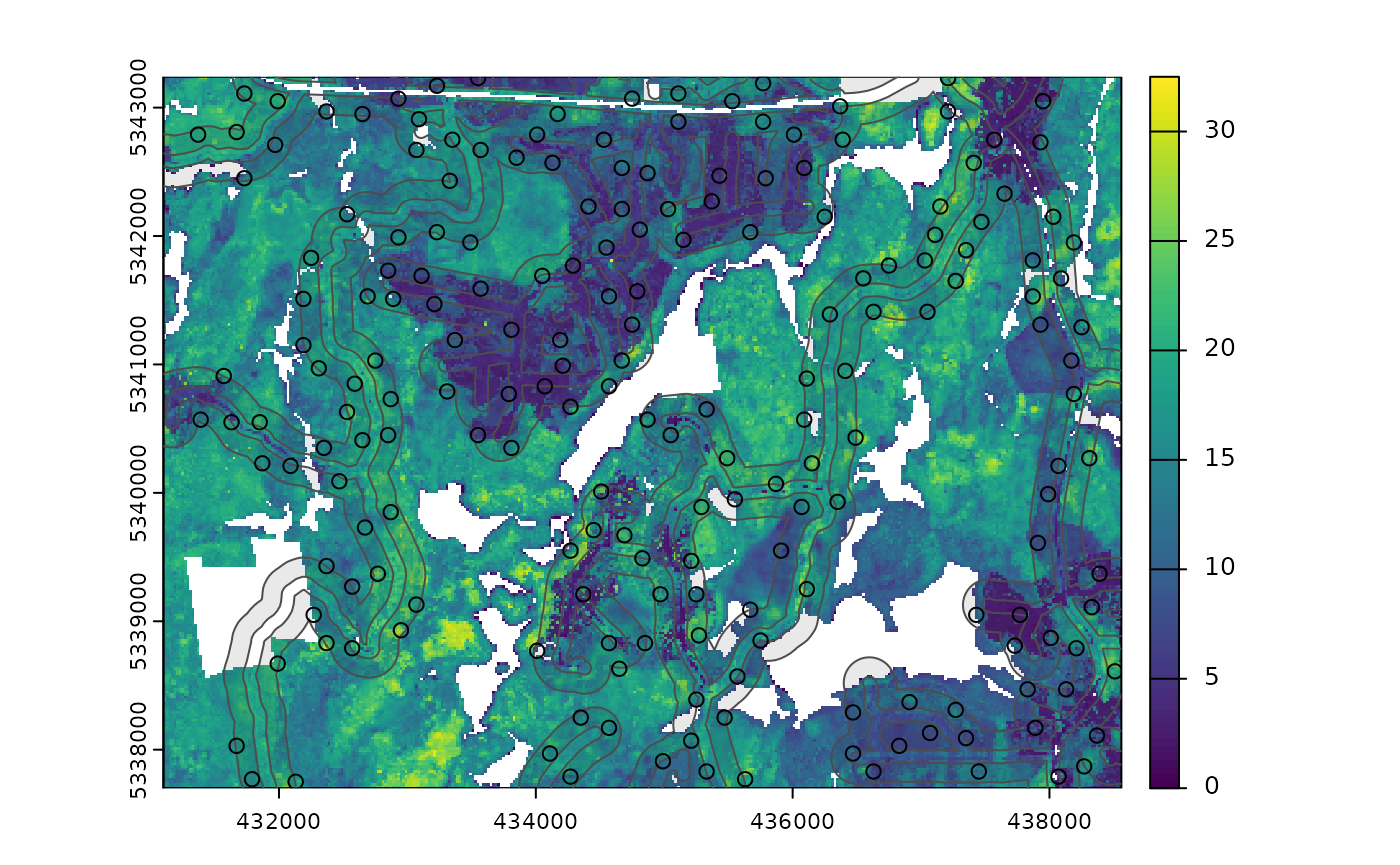#> Simple feature collection with 200 features and 0 fields
#> Geometry type: POINT
#> Dimension:     XY
#> Bounding box:  xmin: 431150 ymin: 5337710 xmax: 438510 ymax: 5343210
#> CRS:           +proj=utm +zone=17 +ellps=GRS80 +towgs84=0,0,0,0,0,0,0 +units=m +no_defs
#> First 10 features:
#>                  geometry
#> 1  POINT (435770 5339810)
#> 2  POINT (432490 5341610)
#> 3  POINT (436750 5338370)
#> 4  POINT (436290 5340170)
#> 5  POINT (436570 5337810)
#> 6  POINT (435390 5340490)
#> 7  POINT (434030 5342910)
#> 8  POINT (435170 5340710)
#> 9  POINT (433410 5342470)
#> 10 POINT (432650 5340450)

## sample_systematic

The sample_systematic() function applies systematic sampling across an area with the cellsize parameter defining the resolution of the tessellation. The tessellation shape can be modified using the square parameter. Assigning TRUE (default) to the square parameter results in a regular grid and assigning FALSE results in a hexagonal grid.

The location of sample units can also be adjusted using the locations parameter, where centers takes the center, corners takes all corners, and random takes a random location within each tessellation. Random start points and translations are applied when the function is called.

#--- perform grid sampling ---#
sample_systematic(raster = sraster, # input sraster
cellsize = 1000, # grid distance
plot = TRUE) # plot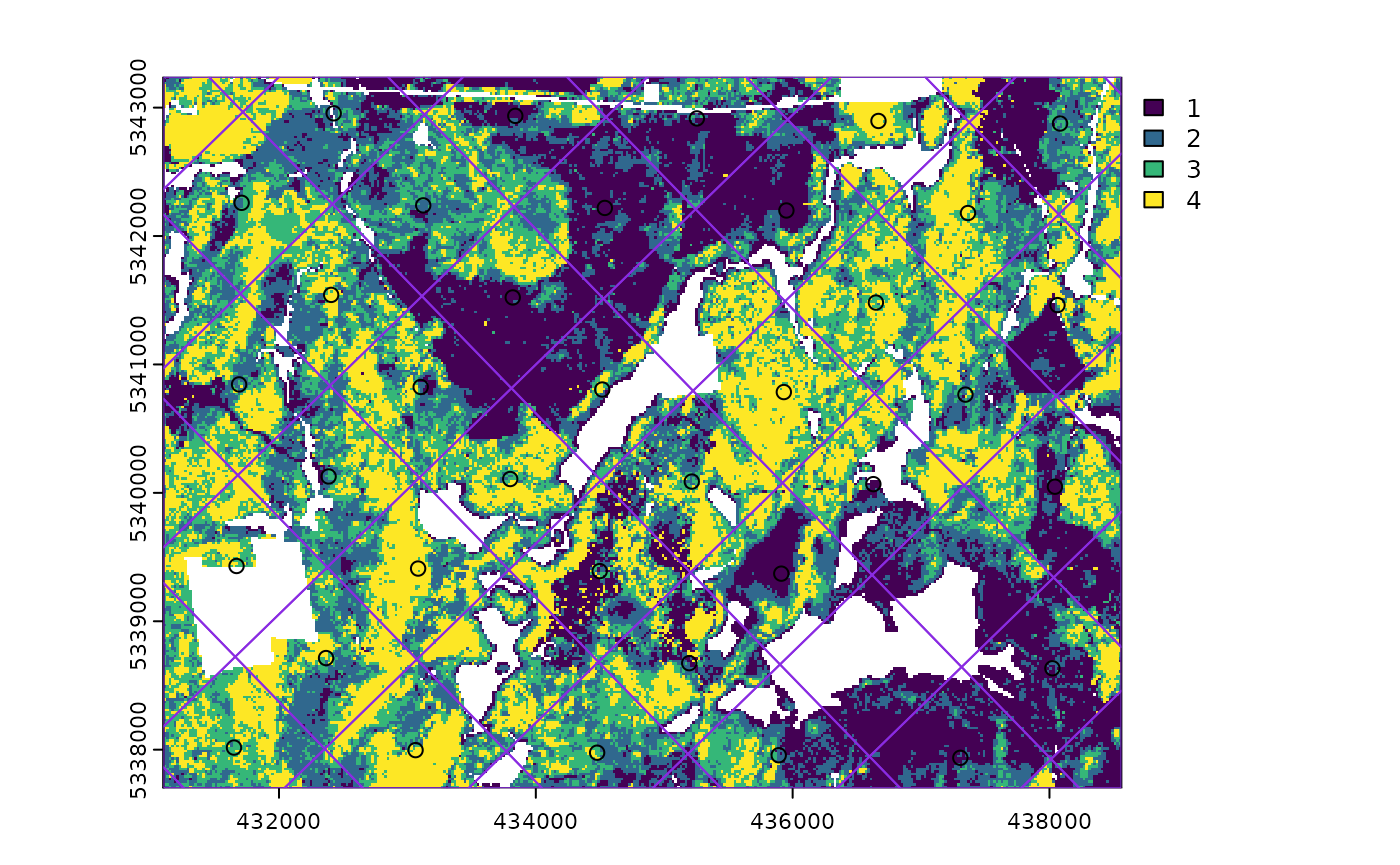#> Simple feature collection with 39 features and 0 fields
#> Geometry type: POINT
#> Dimension:     XY
#> Bounding box:  xmin: 431337.6 ymin: 5337863 xmax: 438547.5 ymax: 5343178
#> CRS:           +proj=utm +zone=17 +ellps=GRS80 +towgs84=0,0,0,0,0,0,0 +units=m +no_defs
#> First 10 features:
#>                    geometry
#> 1  POINT (438547.5 5338114)
#> 2  POINT (437155.8 5337863)
#> 3  POINT (437725.9 5338684)
#> 4  POINT (438296.1 5339506)
#> 5  POINT (436334.2 5338433)
#> 6  POINT (436904.4 5339255)
#> 7  POINT (437474.5 5340076)
#> 8  POINT (438044.7 5340898)
#> 9  POINT (434942.6 5338182)
#> 10 POINT (436082.8 5339825)
#--- perform grid sampling ---#
sample_systematic(raster = sraster, # input sraster
cellsize = 500, # grid distance
square = FALSE, # hexagonal tessellation
location = "random", # randomly sample within tessellation
plot = TRUE) # plot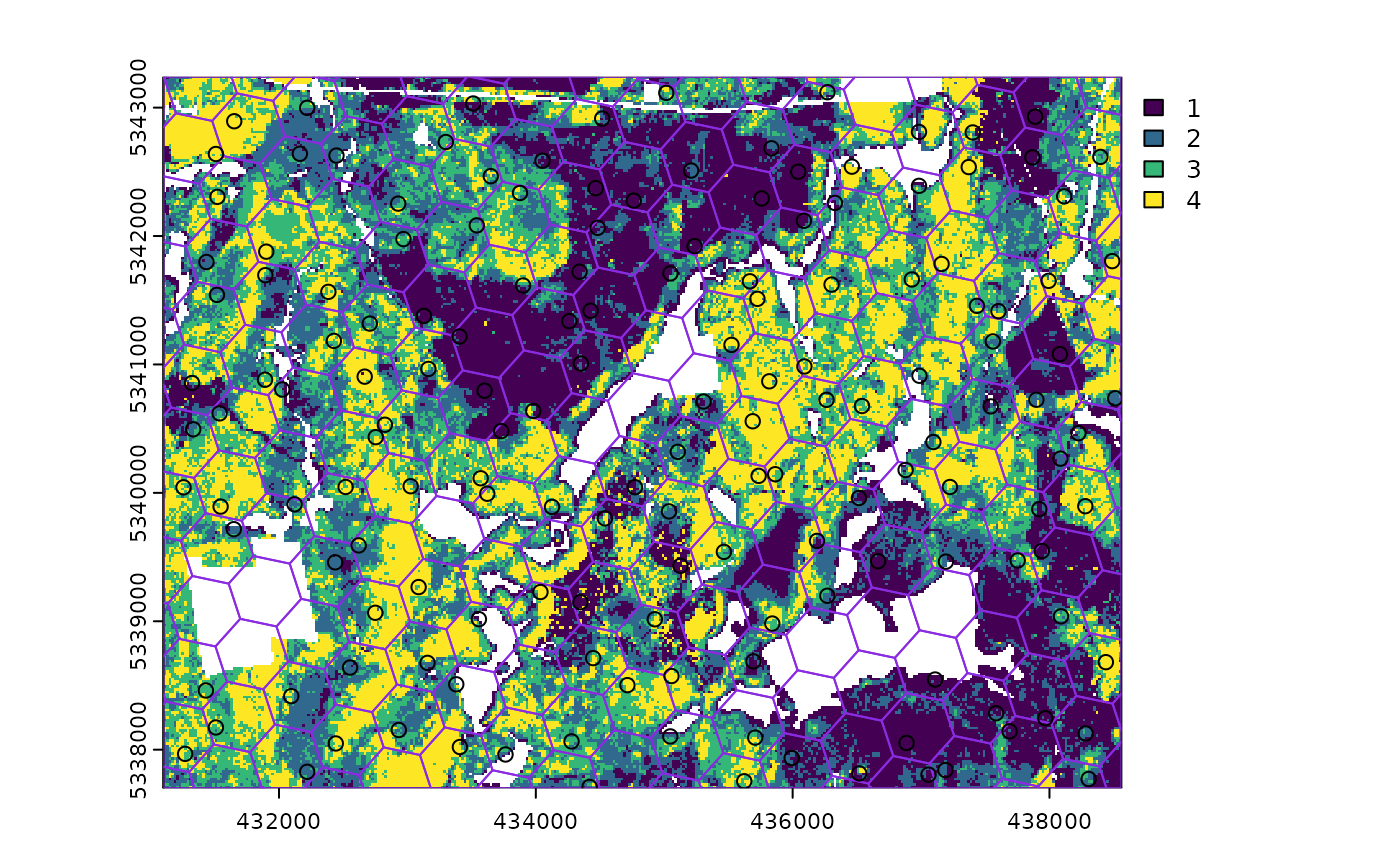#> Simple feature collection with 172 features and 0 fields
#> Geometry type: POINT
#> Dimension:     XY
#> Bounding box:  xmin: 431147.8 ymin: 5337706 xmax: 438522.9 ymax: 5343229
#> CRS:           +proj=utm +zone=17 +ellps=GRS80 +towgs84=0,0,0,0,0,0,0 +units=m +no_defs
#> First 10 features:
#>                    geometry
#> 1  POINT (438501.2 5343010)
#> 2  POINT (437320.4 5342939)
#> 3  POINT (438076.1 5343063)
#> 4  POINT (435201.1 5343229)
#> 5  POINT (436044.5 5343130)
#> 6  POINT (436636.3 5342848)
#> 7  POINT (437665.5 5342934)
#> 8  POINT (438418.1 5342554)
#> 9  POINT (434717.8 5343109)
#> 10 POINT (435345.2 5342799)
sample_systematic(raster = sraster, # input sraster
cellsize = 500, # grid distance
access = access, # define access road network
buff_outer = 200, # outer buffer - no sample units further than this distance from road
square = FALSE, # hexagonal tessellation
location = "corners", # take corners instead of centers
plot = TRUE)#> Simple feature collection with 628 features and 0 fields
#> Geometry type: POINT
#> Dimension:     XY
#> Bounding box:  xmin: 431127 ymin: 5337706 xmax: 438501.7 ymax: 5343224
#> CRS:           +proj=utm +zone=17 +ellps=GRS80 +towgs84=0,0,0,0,0,0,0 +units=m +no_defs
#> First 10 features:
#>                    geometry
#> 1  POINT (431324.9 5342738)
#> 2    POINT (431127 5342528)
#> 3  POINT (431720.8 5343158)
#> 4    POINT (431127 5342528)
#> 5  POINT (431324.9 5342738)
#> 6  POINT (431720.8 5343158)
#> 7  POINT (431803.8 5342882)
#> 8  POINT (431605.9 5342672)
#> 9  POINT (431324.9 5342738)
#> 10   POINT (431127 5342528)

## sample_strat

The sample_strat() contains two methods to perform sampling:

• "Queinnec" - Hierarchical sampling using a focal window to isolate contiguous groups of stratum pixels, which was originally developed by Martin Queinnec.

• "random" - Traditional stratified random sampling. This method ignores much of the functionality of the algorithm to allow users the capability to use standard stratified random sampling approaches without the use of a focal window to locate contiguous stratum cells.

### method = "Queinnec"

Queinnec, M., White, J. C., & Coops, N. C. (2021). Comparing airborne and spaceborne photon-counting LiDAR canopy structural estimates across different boreal forest types. Remote Sensing of Environment, 262(August 2020), 112510.

This algorithm uses moving window (wrow and wcol parameters) to filter the input sraster to prioritize sample unit allocation to where stratum pixels are spatially grouped, rather than dispersed individuals across the landscape.

Sampling is performed using 2 rules:

• Rule 1 - Sample within spatially grouped stratum pixels. Moving window defined by wrow and wcol.

• Rule 2 - If no additional sample units exist to satisfy desired sample size(nSamp), individual stratum pixels are sampled.

The rule applied to a select each sample unit is defined in the rule attribute of output samples. We give a few examples below:

#--- perform stratified sampling random sampling ---#
sample_strat(sraster = sraster, # input sraster
nSamp = 200) # desired sample size # plot
#> Simple feature collection with 200 features and 3 fields
#> Geometry type: POINT
#> Dimension:     XY
#> Bounding box:  xmin: 431130 ymin: 5337750 xmax: 438530 ymax: 5343230
#> CRS:           NA
#> First 10 features:
#>    strata type  rule               geometry
#> x       1  new rule1 POINT (435270 5342170)
#> x1      1  new rule2 POINT (434570 5341570)
#> x2      1  new rule2 POINT (435730 5342250)
#> x3      1  new rule2 POINT (434550 5342310)
#> x4      1  new rule2 POINT (434470 5340930)
#> x5      1  new rule2 POINT (437810 5338110)
#> x6      1  new rule2 POINT (436790 5338630)
#> x7      1  new rule2 POINT (438450 5338370)
#> x8      1  new rule2 POINT (438290 5340930)
#> x9      1  new rule2 POINT (437330 5338470)

In some cases, users might want to include an existing sample within the algorithm. In order to adjust the total number of sample units needed per stratum to reflect those already present in existing, we can use the intermediate function extract_strata().

This function uses the sraster and existing sample units and extracts the stratum for each. These sample units can be included within sample_strat(), which adjusts total sample units required per class based on representation in existing.

#--- extract strata values to existing samples ---#
e.sr <- extract_strata(sraster = sraster, # input sraster
existing = existing) # existing samples to add strata value to

TIP!

sample_strat() requires the sraster input to have an attribute named strata and will give an error if it doesn’t.

sample_strat(sraster = sraster, # input sraster
nSamp = 200, # desired sample size
access = access, # define access road network
existing = e.sr, # existing sample with strata values
mindist = 200, # minimum distance sample units must be apart from one another
buff_inner = 50, # inner buffer - no sample units within this distance from road
buff_outer = 200, # outer buffer - no sample units further than this distance from road
plot = TRUE) # plot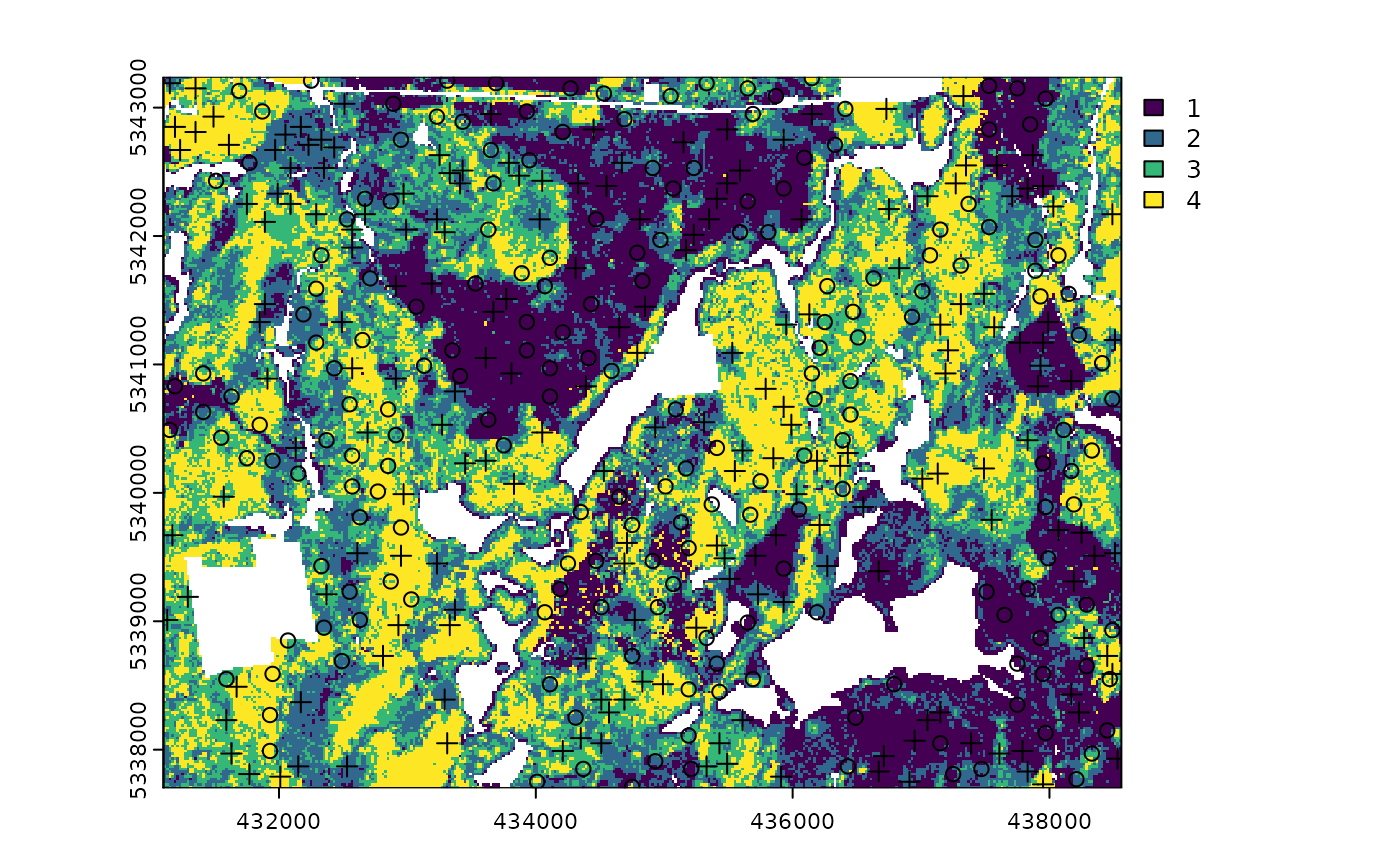#> Simple feature collection with 400 features and 3 fields
#> Geometry type: POINT
#> Dimension:     XY
#> Bounding box:  xmin: 431110 ymin: 5337710 xmax: 438550 ymax: 5343230
#> CRS:           NA
#> First 10 features:
#>    strata     type     rule               geometry
#> 1       1 existing existing POINT (434050 5340830)
#> 2       1 existing existing POINT (434670 5341810)
#> 3       1 existing existing POINT (435250 5342010)
#> 4       1 existing existing POINT (432910 5341730)
#> 5       1 existing existing POINT (434330 5341730)
#> 6       1 existing existing POINT (436750 5339050)
#> 7       1 existing existing POINT (436910 5338290)
#> 8       1 existing existing POINT (435410 5341970)
#> 9       1 existing existing POINT (434210 5342630)
#> 10      1 existing existing POINT (433190 5343210)

The code in the example above defined the mindist parameter, which specifies the minimum euclidean distance that new sample units must be apart from one another.

Notice that the sample units have type and rule attributes which outline whether they are existing or new, and whether rule1 or rule2 were used to select them. If type is existing (a user provided existing sample), rule will be existing as well as seen above.

sample_strat(sraster = sraster, # input
nSamp = 200, # desired sample size
access = access, # define access road network
existing = e.sr, # existing samples with strata values
include = TRUE, # include existing sample in nSamp total
buff_outer = 200, # outer buffer - no samples further than this distance from road
plot = TRUE) # plot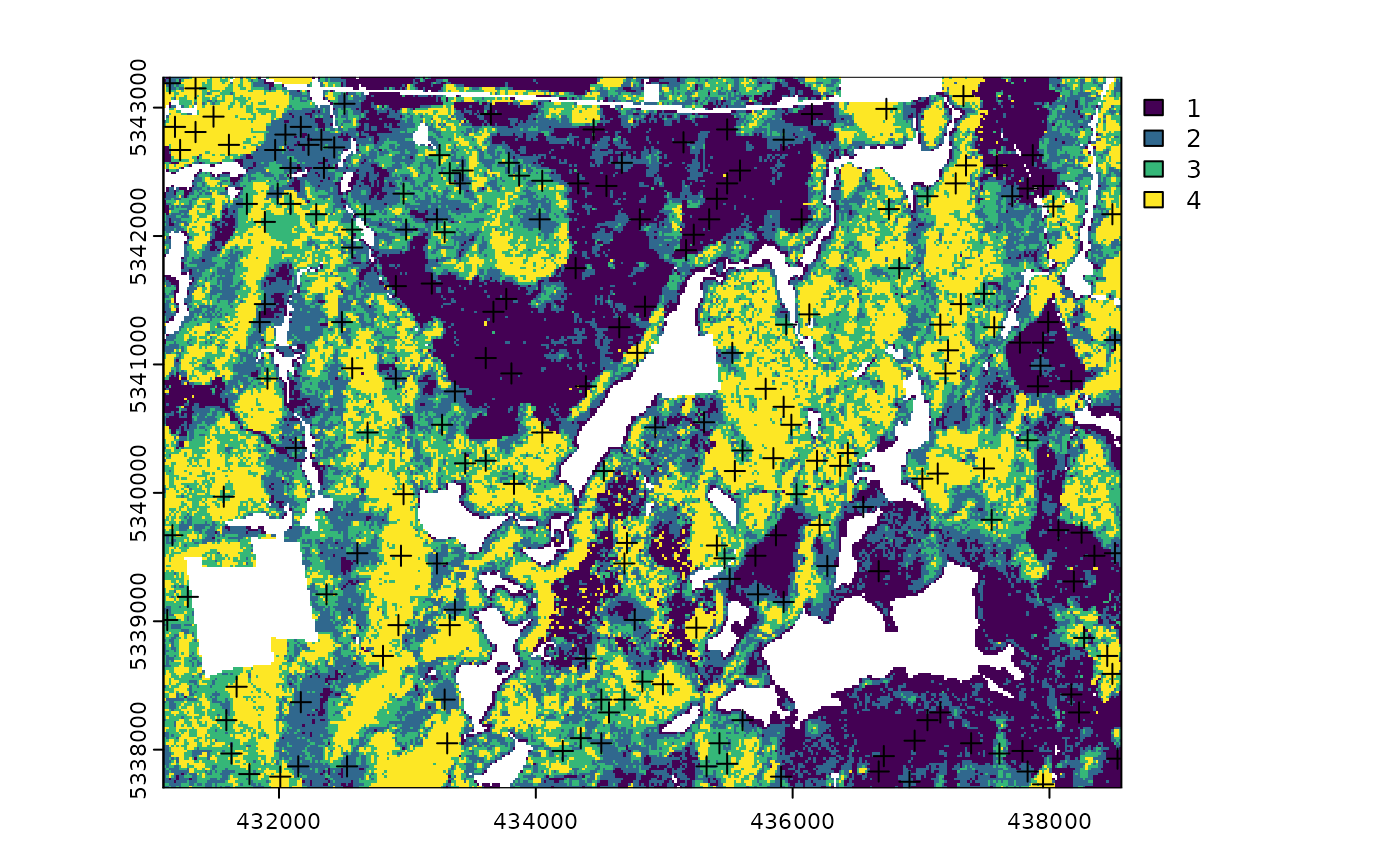#> Simple feature collection with 200 features and 3 fields
#> Geometry type: POINT
#> Dimension:     XY
#> Bounding box:  xmin: 431130 ymin: 5337710 xmax: 438550 ymax: 5343230
#> CRS:           NA
#> First 10 features:
#>    strata     type     rule               geometry
#> 1       1 existing existing POINT (434050 5340830)
#> 2       1 existing existing POINT (434670 5341810)
#> 3       1 existing existing POINT (435250 5342010)
#> 4       1 existing existing POINT (432910 5341730)
#> 5       1 existing existing POINT (434330 5341730)
#> 6       1 existing existing POINT (436750 5339050)
#> 7       1 existing existing POINT (436910 5338290)
#> 8       1 existing existing POINT (435410 5341970)
#> 9       1 existing existing POINT (434210 5342630)
#> 10      1 existing existing POINT (433190 5343210)

The include parameter determines whether existing sample units should be included in the total sample size defined by nSamp. By default, the include parameter is set as FALSE.

### method = "random

Stratified random sampling with equal probability for all cells (using default algorithm values for mindist and no use of access functionality). In essence this method perform the sample_srs algorithm for each stratum separately to meet the specified sample size.

#--- perform stratified sampling random sampling ---#
sample_strat(sraster = sraster, # input sraster
method = "random", #stratified random sampling
nSamp = 200, # desired sample size
plot = TRUE) # plot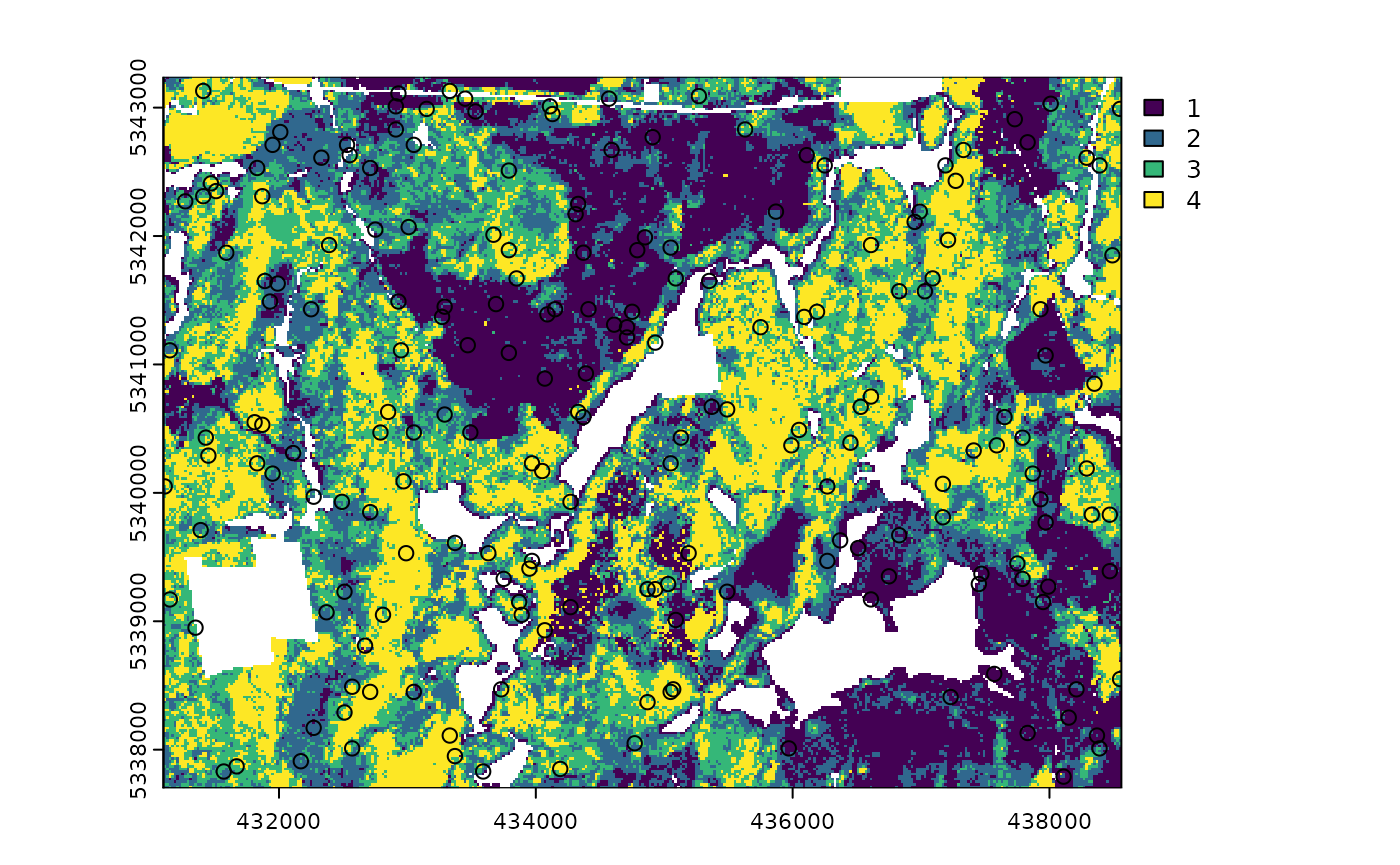#> Simple feature collection with 200 features and 1 field
#> Geometry type: POINT
#> Dimension:     XY
#> Bounding box:  xmin: 431230 ymin: 5337810 xmax: 438550 ymax: 5343210
#> Projected CRS: UTM Zone 17, Northern Hemisphere
#> First 10 features:
#>    strata               geometry
#> 1       1 POINT (436490 5338150)
#> 2       1 POINT (438430 5338210)
#> 3       1 POINT (432790 5342410)
#> 4       1 POINT (434470 5342350)
#> 5       1 POINT (434690 5342470)
#> 6       1 POINT (437110 5338490)
#> 7       1 POINT (435650 5339130)
#> 8       1 POINT (434210 5338910)
#> 9       1 POINT (438070 5341270)
#> 10      1 POINT (434450 5341590)

## sample_sys_strat

sample_sys_strat() function implements systematic stratified sampling on and sraster. This function uses the same functionality as sample_systematic() but takes an sraster as input and performs sampling on each stratum iteratively.

#--- perform grid sampling on each stratum separately ---#
sample_sys_strat(
sraster = sraster, # input sraster
cellsize = 1000, # grid size
plot = TRUE # plot output
)
#> Processing strata : 1
#> Processing strata : 2
#> Processing strata : 3
#> Processing strata : 4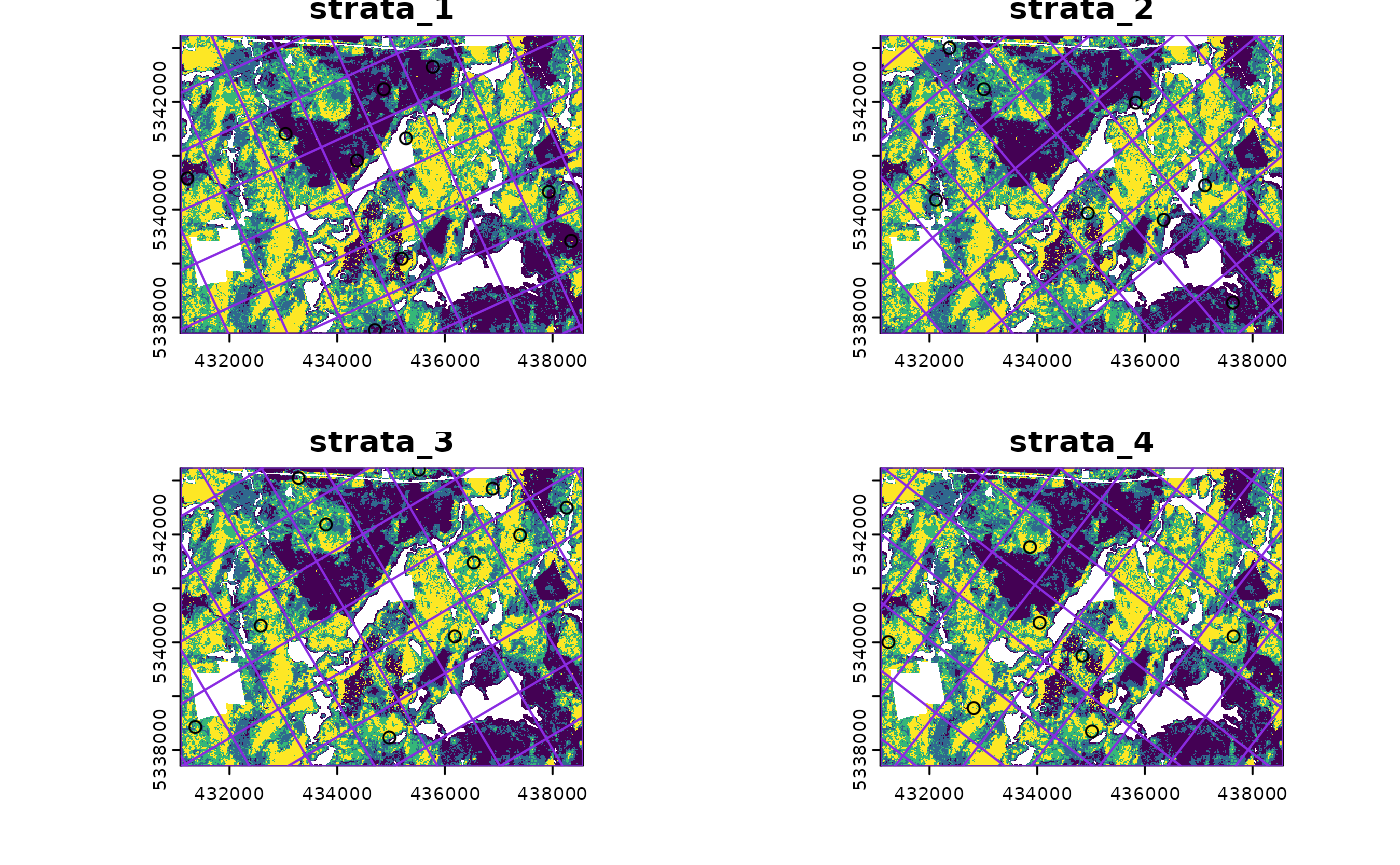#> Simple feature collection with 28 features and 1 field
#> Geometry type: POINT
#> Dimension:     XY
#> Bounding box:  xmin: 431166.2 ymin: 5337703 xmax: 438300.8 ymax: 5343003
#> Projected CRS: UTM Zone 17, Northern Hemisphere
#> First 10 features:
#>    strata                 geometry
#> 1       1 POINT (431166.2 5342099)
#> 2       1 POINT (432333.2 5339523)
#> 3       1 POINT (434326.2 5341978)
#> 4       1 POINT (433973.8 5341042)
#> 5       1 POINT (435614.4 5342561)
#> 6       1 POINT (434909.7 5340690)
#> 7       1 POINT (437838.5 5342793)
#> 8       1 POINT (436076.7 5338113)
#> 9       1 POINT (438069.6 5340568)
#> 10      1 POINT (437012.5 5337761)

Just like with sample_systematic() we can specify where we want our samples to fall within our tessellations. We specify location = "corners" below. Note that the tesselations are all saved to a list file when details = TRUE should the user want to save them.

sample_sys_strat(
sraster = sraster, # input sraster
cellsize = 500, # grid size
square = FALSE, # hexagon tessellation
location = "corners", # samples on tessellation corners
plot = TRUE # plot output
)
#> Processing strata : 1
#> Processing strata : 2
#> Processing strata : 3
#> Processing strata : 4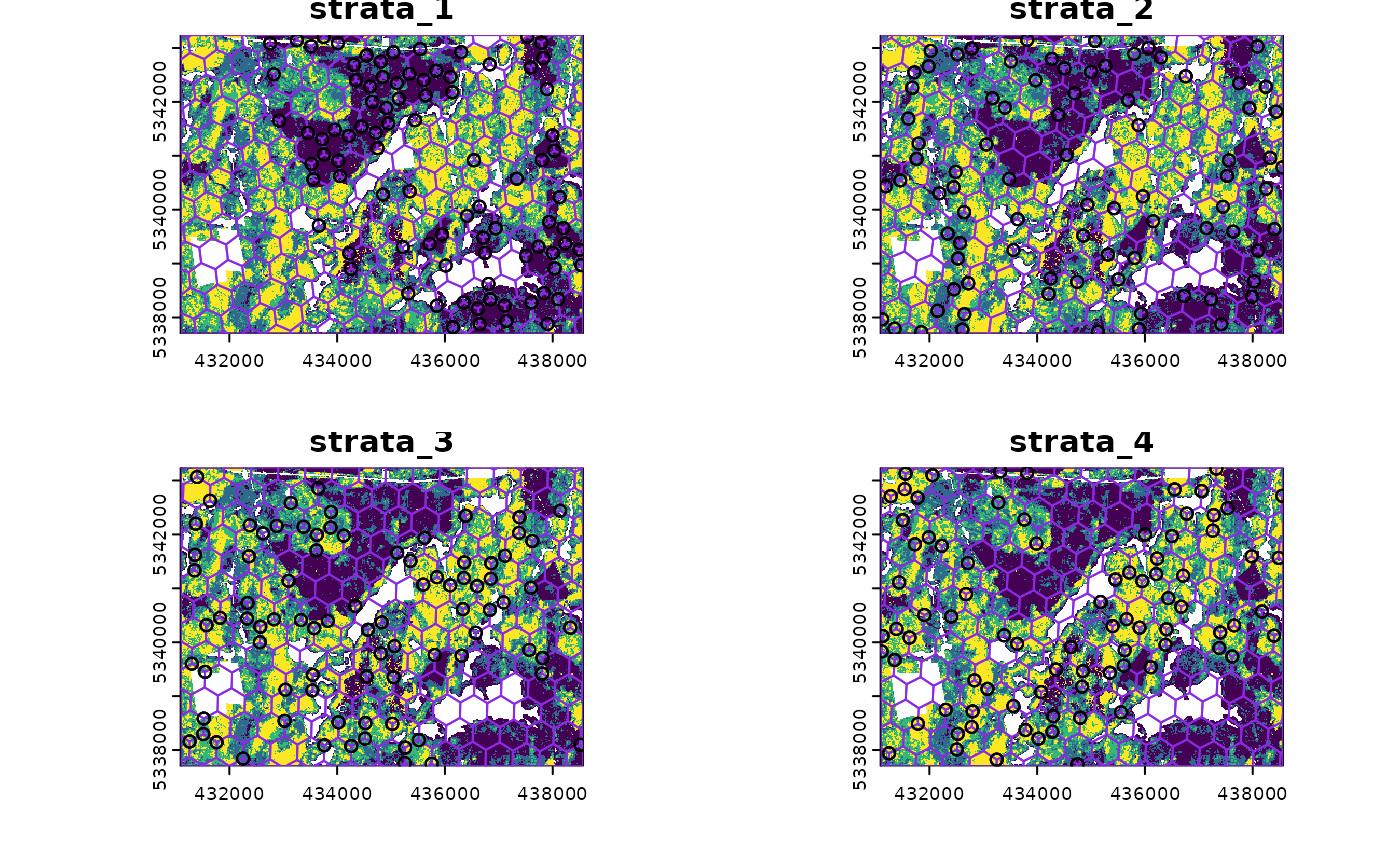#> Simple feature collection with 1176 features and 1 field
#> Geometry type: POINT
#> Dimension:     XY
#> Bounding box:  xmin: 431108.8 ymin: 5337752 xmax: 438536.4 ymax: 5343229
#> Projected CRS: UTM Zone 17, Northern Hemisphere
#> First 10 features:
#>    strata                 geometry
#> 1       1   POINT (431305 5340713)
#> 2       1   POINT (431305 5340713)
#> 3       1 POINT (431242.2 5342035)
#> 4       1   POINT (431305 5340713)
#> 5       1 POINT (431242.2 5342035)
#> 6       1 POINT (431383.3 5342514)
#> 7       1 POINT (431242.2 5342035)
#> 8       1 POINT (431383.3 5342514)
#> 9       1 POINT (431660.2 5342433)
#> 10      1 POINT (431660.2 5342433)

This sampling approach could be especially useful incombination with strat_poly() to ensure consistency of sampling accross specific management units.

#--- read polygon coverage ---#
poly <- system.file("extdata", "inventory_polygons.shp", package = "sgsR")
#> Reading layer inventory_polygons' from data source
#>   /home/runner/work/_temp/Library/sgsR/extdata/inventory_polygons.shp'
#>   using driver ESRI Shapefile'
#> Simple feature collection with 632 features and 3 fields
#> Geometry type: MULTIPOLYGON
#> Dimension:     XY
#> Bounding box:  xmin: 431100 ymin: 5337700 xmax: 438560 ymax: 5343240
#> Projected CRS: UTM_Zone_17_Northern_Hemisphere

#--- stratify polygon coverage ---#
#--- specify polygon attribute to stratify ---#
attribute <- "NUTRIENTS"

#--- specify features within attribute & how they should be grouped ---#
#--- as a single vector ---#
features <- c("poor", "rich", "medium")

#--- get polygon stratification ---#
srasterpoly <- strat_poly(
poly = fri,
attribute = attribute,
features = features,
raster = sraster
)

#--- systematatic stratified sampling for each stratum ---#
sample_sys_strat(
sraster = srasterpoly, # input sraster from strat_poly()
cellsize = 1000, # grid size
square = FALSE, # hexagon tessellation
location = "random", # randomize plot location
plot = TRUE # plot output
)
#> Processing strata : 1
#> Processing strata : 2
#> Processing strata : 3
#> Simple feature collection with 37 features and 1 field
#> Geometry type: POINT
#> Dimension:     XY
#> Bounding box:  xmin: 431204.7 ymin: 5338050 xmax: 438214.5 ymax: 5343118
#> Projected CRS: UTM Zone 17, Northern Hemisphere
#> First 10 features:
#>    strata                 geometry
#> 1       1 POINT (431204.7 5339907)
#> 2       1 POINT (431479.5 5342178)
#> 3       1 POINT (432358.3 5342983)
#> 4       1 POINT (432356.8 5341973)
#> 5       1 POINT (432941.8 5343042)
#> 6       1   POINT (433050 5342121)
#> 7       1 POINT (433498.3 5341145)
#> 8       1 POINT (434210.3 5342764)
#> 9       1   POINT (433940 5338654)
#> 10      1 POINT (434652.7 5341677)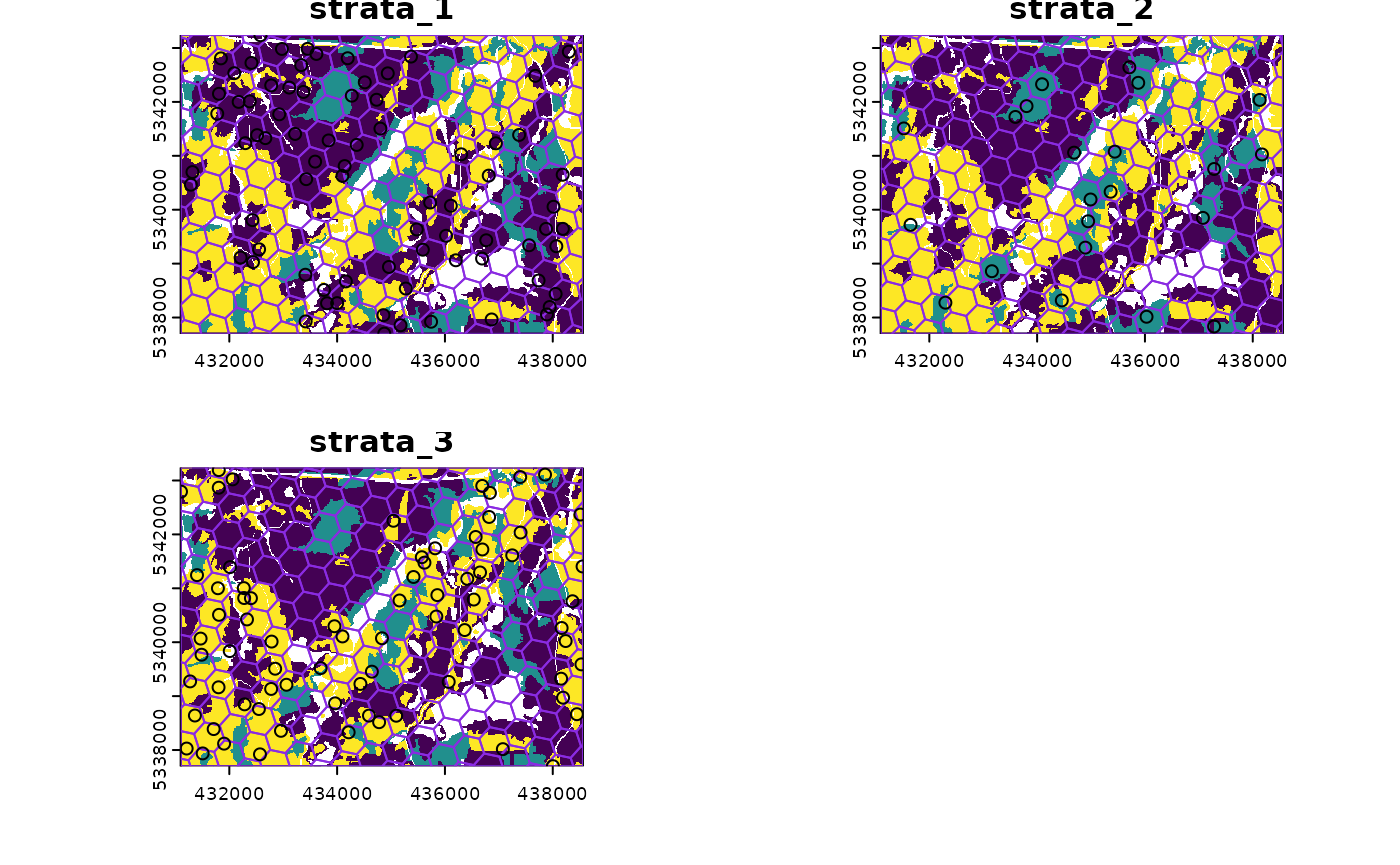## sample_nc

sample_nc() function implements the Nearest Centroid sampling algorithm described in Melville & Stone (2016). The algorithm uses kmeans clustering where the number of clusters (centroids) is equal to the desired sample size (nSamp).

Cluster centers are located, which then prompts the nearest neighbour mraster pixel for each cluster to be selected (assuming default k parameter). These nearest neighbours are the output sample units.

#--- perform simple random sampling ---#
sample_nc(mraster = mraster, # input
nSamp = 25, # desired sample size
plot = TRUE)
#> K-means being performed on 3 layers with 25 centers.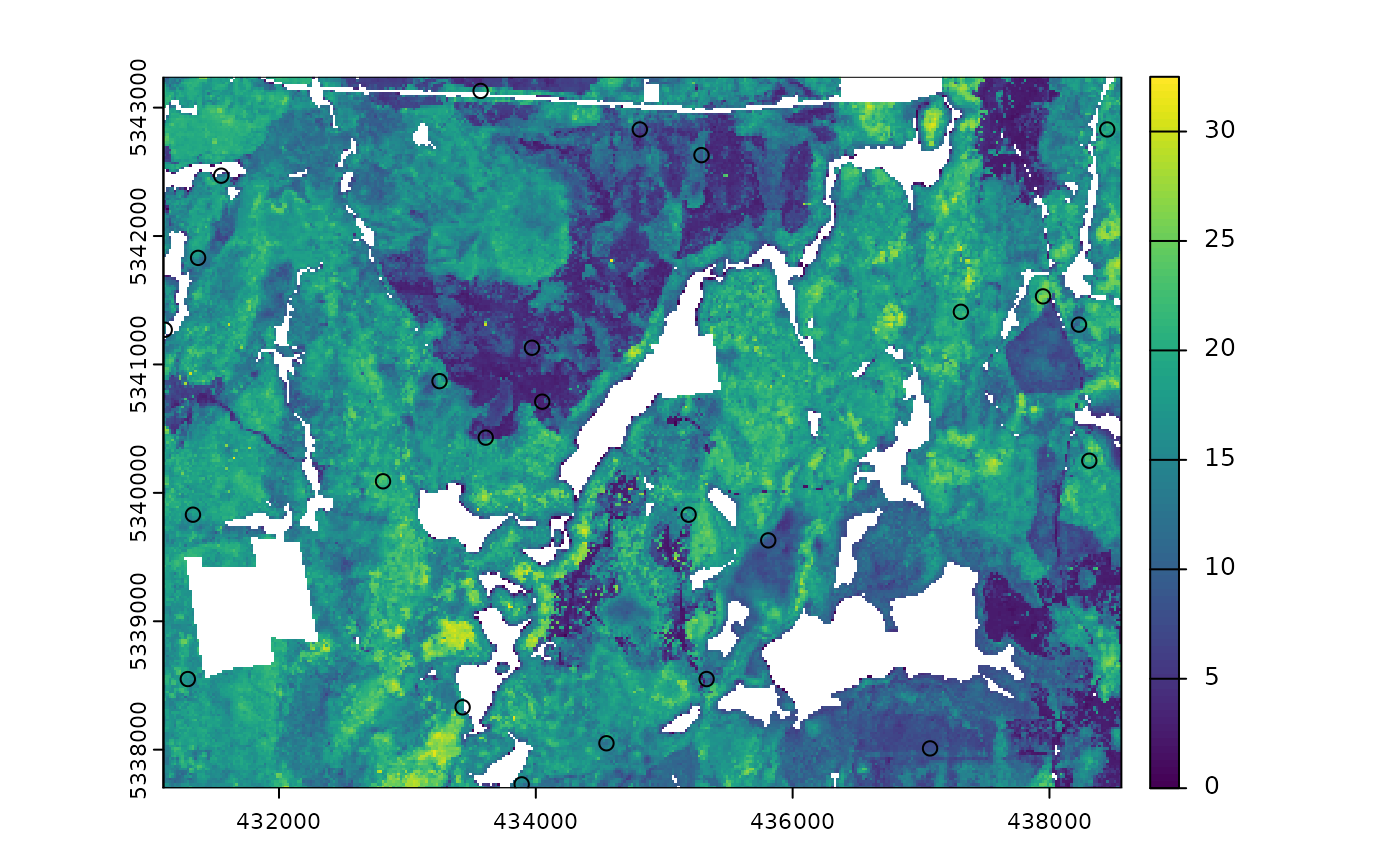#> Simple feature collection with 25 features and 4 fields
#> Geometry type: POINT
#> Dimension:     XY
#> Bounding box:  xmin: 431210 ymin: 5338110 xmax: 438510 ymax: 5343190
#> CRS:           +proj=utm +zone=17 +ellps=GRS80 +towgs84=0,0,0,0,0,0,0 +units=m +no_defs
#> First 10 features:
#>        zq90 pzabove2  zsd kcenter               geometry
#> 59102 21.90     92.3 5.25       1 POINT (434450 5340070)
#> 8226  20.50     84.8 6.01       2 POINT (431490 5342790)
#> 21282 10.00     68.9 2.58       3 POINT (431510 5342090)
#> 19838 17.70     93.3 3.43       4 POINT (432470 5342170)
#> 64549 15.30     95.6 2.70       5 POINT (431490 5339770)
#> 47146  3.07      6.7 0.60       6 POINT (434050 5340710)
#> 859    6.19     60.5 1.45       7 POINT (433350 5343190)
#> 67385  8.54     40.4 2.25       8 POINT (435990 5339630)
#> 49010  4.55     30.7 1.02       9 POINT (434030 5340610)
#> 63615 19.50     20.9 6.20      10 POINT (435190 5339830)

Altering the k parameter leads to a multiplicative increase in output sample units where total output samples = $$nSamp * k$$.

#--- perform simple random sampling ---#
samples <- sample_nc(mraster = mraster, # input
k = 2, # number of nearest neighbours to take for each kmeans center
nSamp = 25, # desired sample size
plot = TRUE)
#> K-means being performed on 3 layers with 25 centers.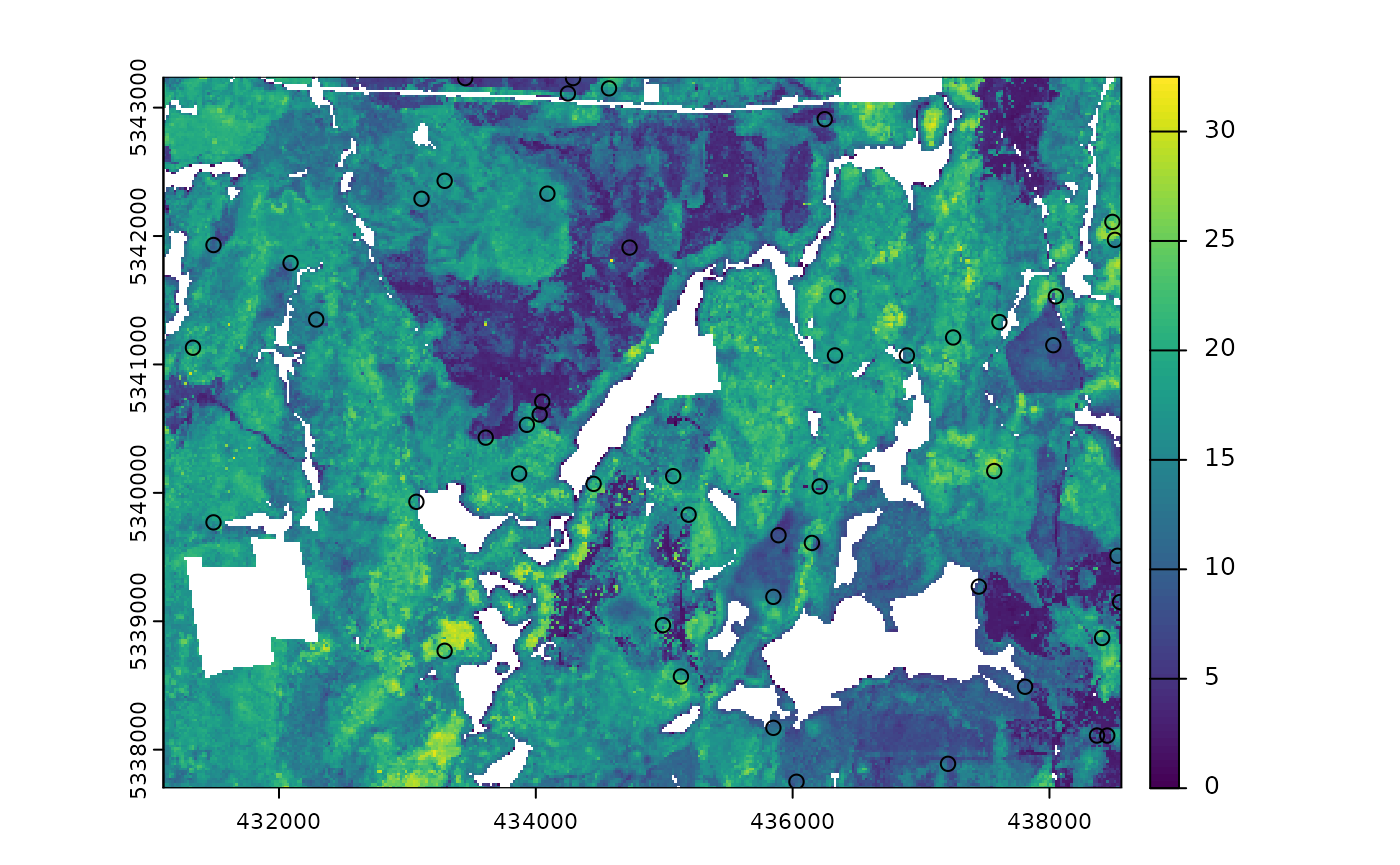#--- total samples = nSamp * k (25 * 2) = 50 ---#
nrow(samples)
#>  50

Visualizing what the kmeans centers and sample units looks like is possible when using details = TRUE. The $kplot output provides a quick visualization of where the centers are based on a scatter plot of the first 2 layers in mraster. Notice that the centers are well distributed in covariate space and chosen sample units are the closest pixels to each center (nearest neighbours). #--- perform simple random sampling with details ---# details <- sample_nc(mraster = mraster, # input nSamp = 25, # desired sample number details = TRUE) #> K-means being performed on 3 layers with 25 centers. #--- plot ggplot output ---# details$kplot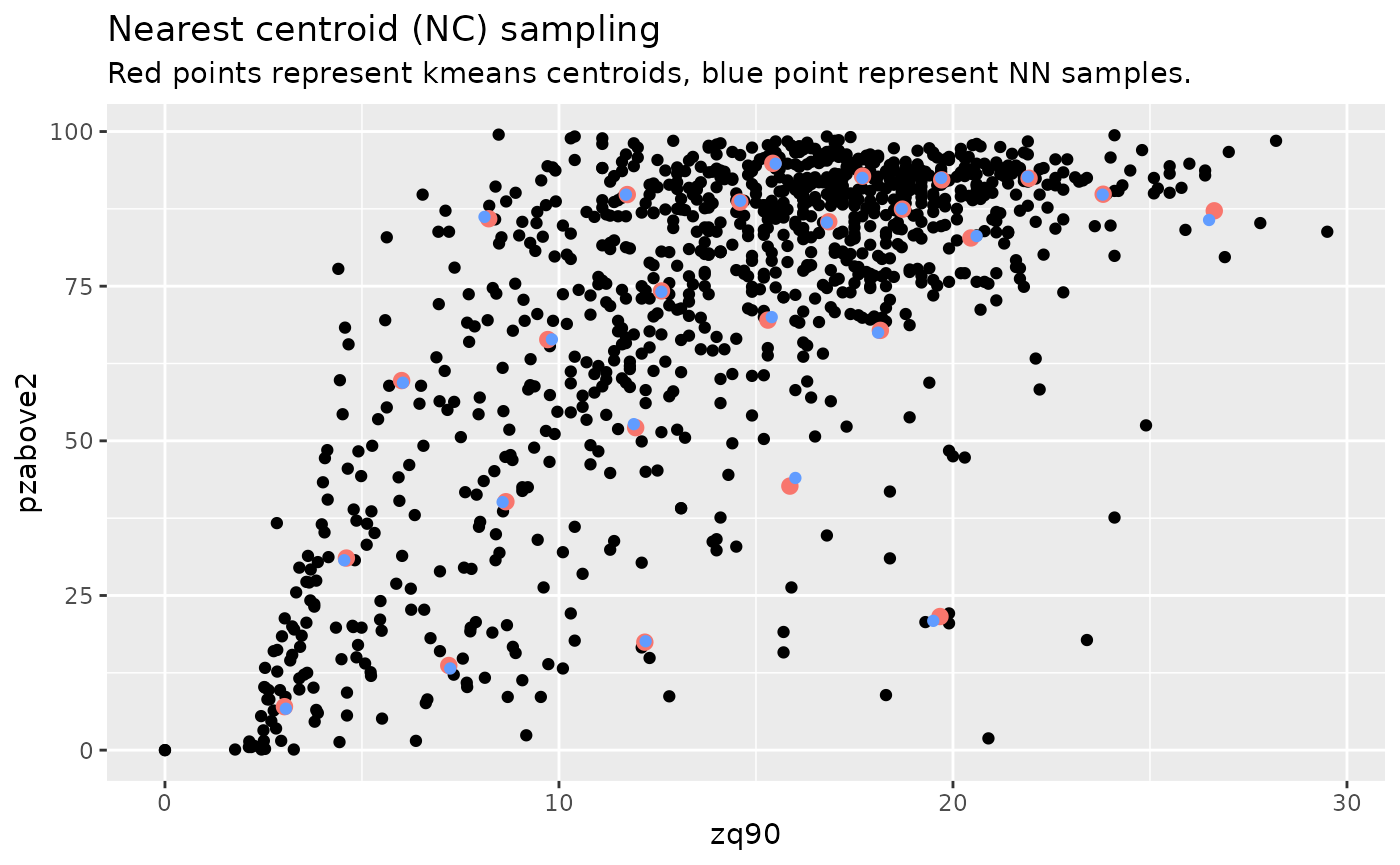## sample_clhs

sample_clhs() function implements conditioned Latin hypercube (clhs) sampling methodology from the clhs package.

TIP!

A number of other functions in the sgsR package help to provide guidance on clhs sampling including calculate_pop() and calculate_lhsOpt(). Check out these functions to better understand how sample numbers could be optimized.

The syntax for this function is similar to others shown above, although parameters like iter, which define the number of iterations within the Metropolis-Hastings process are important to consider. In these examples we use a low iter value for efficiency. Default values for iter within the clhs package are 10,000.

sample_clhs(mraster = mraster, # input
nSamp = 200, # desired sample size
plot = TRUE, # plot
iter = 100) # number of iterations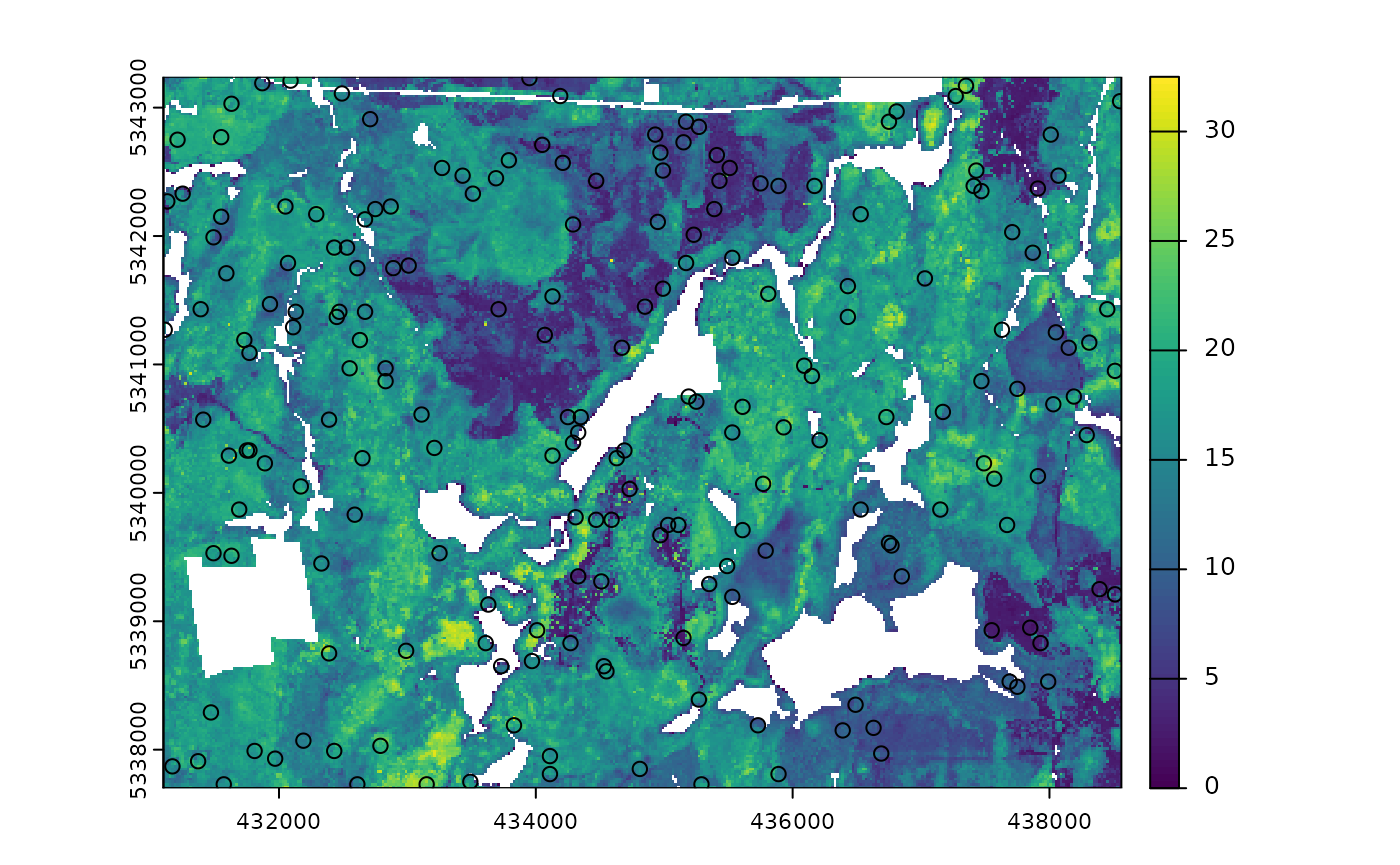The cost parameter defines the mraster covariate, which is used to constrain the clhs sampling. An example could be the distance a pixel is from road access (e.g. from calculate_distance() see example below), terrain slope, the output from calculate_coobs(), or many others.

#--- cost constrained examples ---#
#--- calculate distance to access layer for each pixel in mr ---#
mr.c <- calculate_distance(raster = mraster, # input
access = access, # define access road network
plot = TRUE) # plot
#>
|---------|---------|---------|---------|
=========================================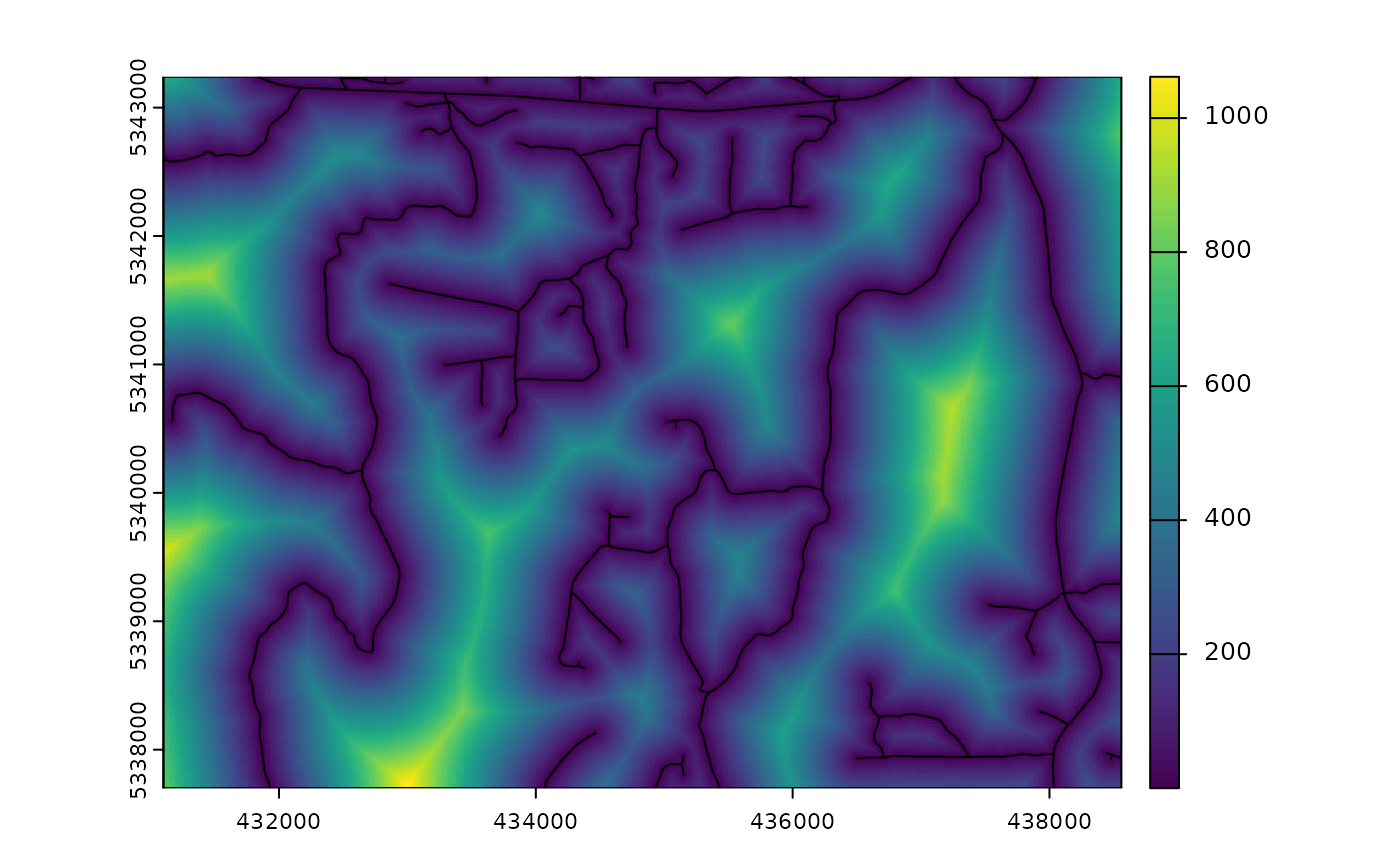sample_clhs(mraster = mr.c, # input
nSamp = 250, # desired sample size
iter = 100, # number of iterations
cost = "dist2access", # cost parameter - name defined in calculate_distance()
plot = TRUE) # plot## sample_balanced

The sample_balanced() algorithm performs a balanced sampling methodology from the stratifyR / SamplingBigData packages.

sample_balanced(mraster = mraster, # input
nSamp = 200, # desired sample size
plot = TRUE) # plot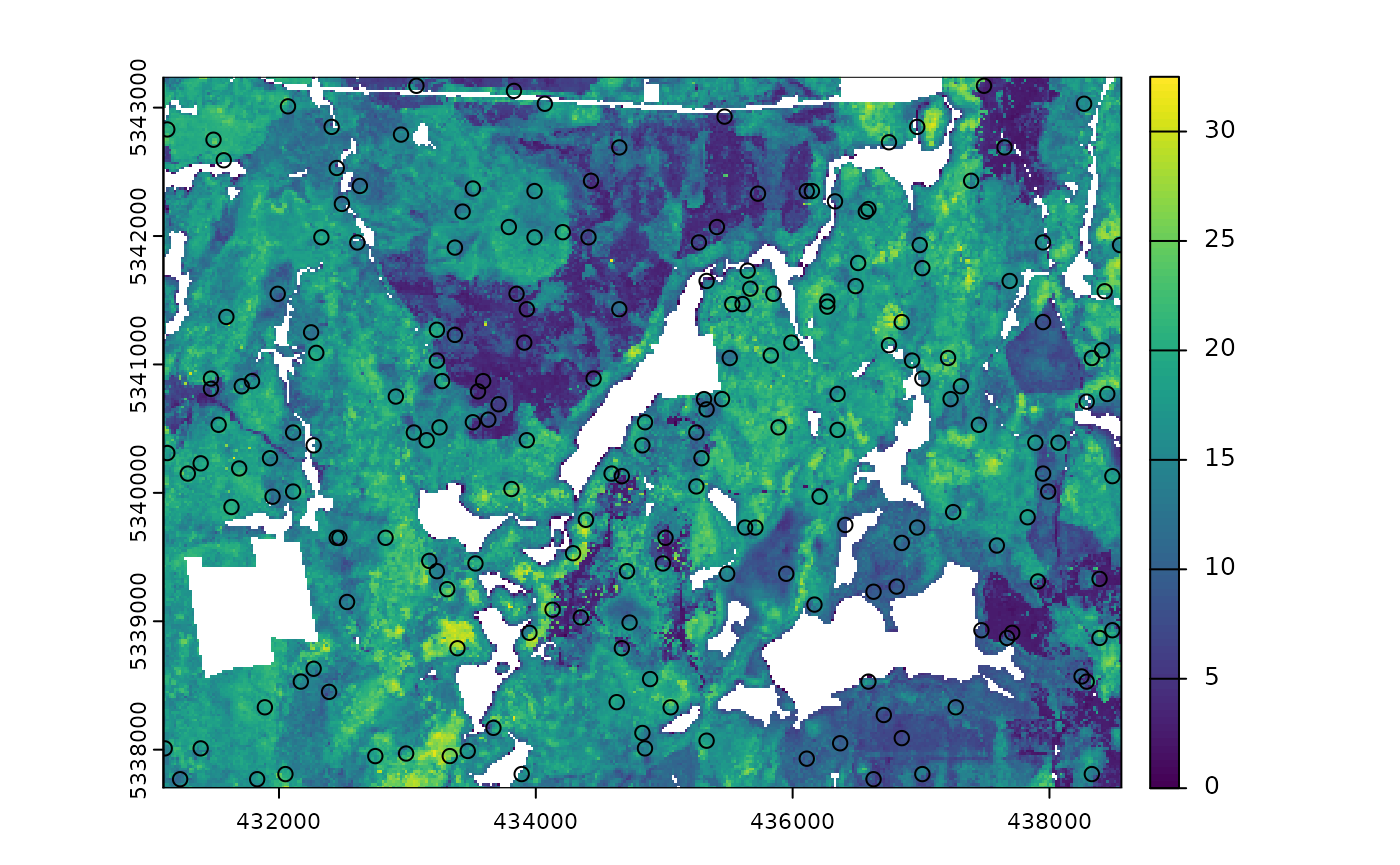#> Simple feature collection with 200 features and 0 fields
#> Geometry type: POINT
#> Dimension:     XY
#> Bounding box:  xmin: 431110 ymin: 5337770 xmax: 438550 ymax: 5343190
#> CRS:           +proj=utm +zone=17 +ellps=GRS80 +towgs84=0,0,0,0,0,0,0 +units=m +no_defs
#> First 10 features:
#>                  geometry
#> 1  POINT (437630 5343190)
#> 2  POINT (434470 5343170)
#> 3  POINT (437850 5343170)
#> 4  POINT (432170 5343130)
#> 5  POINT (437650 5343130)
#> 6  POINT (434010 5343090)
#> 7  POINT (432090 5343010)
#> 8  POINT (438050 5343010)
#> 9  POINT (431670 5342970)
#> 10 POINT (435750 5342950)
sample_balanced(mraster = mraster, # input
nSamp = 100, # desired sample size
algorithm = "lcube", # algorithm type
access = access, # define access road network
buff_inner = 50, # inner buffer - no sample units within this distance from road
buff_outer = 200) # outer buffer - no sample units further than this distance from road
#> Simple feature collection with 100 features and 0 fields
#> Geometry type: POINT
#> Dimension:     XY
#> Bounding box:  xmin: 431270 ymin: 5337810 xmax: 438450 ymax: 5343230
#> CRS:           +proj=utm +zone=17 +ellps=GRS80 +towgs84=0,0,0,0,0,0,0 +units=m +no_defs
#> First 10 features:
#>                  geometry
#> 1  POINT (431270 5340630)
#> 2  POINT (438270 5339270)
#> 3  POINT (432270 5341030)
#> 4  POINT (433470 5341310)
#> 5  POINT (436330 5341630)
#> 6  POINT (434830 5341610)
#> 7  POINT (433750 5340910)
#> 8  POINT (437670 5339250)
#> 9  POINT (436290 5342310)
#> 10 POINT (433010 5338970)

## sample_ahels

The sample_ahels() function performs the adapted Hypercube Evaluation of a Legacy Sample (ahels) algorithm usingexisting sample data and an mraster. New sample units are allocated based on quantile ratios between the existing sample and mraster covariate dataset.

This algorithm was adapted from that presented in the paper below, which we highly recommend.

Malone BP, Minansy B, Brungard C. 2019. Some methods to improve the utility of conditioned Latin hypercube sampling. PeerJ 7:e6451 DOI 10.7717/peerj.6451

This algorithm:

1. Determines the quantile distributions of existing sample units and mraster covariates.

2. Determines quantiles where there is a disparity between sample units and covariates.

3. Prioritizes sampling within those quantile to improve representation.

To use this function, user must first specify the number of quantiles (nQuant) followed by either the nSamp (total number of desired sample units to be added) or the threshold (sampling ratio vs. covariate coverage ratio for quantiles - default is 0.9) parameters.

#--- remove type variable from existing  - causes plotting issues ---#

existing <- existing %>% select(-type)

sample_ahels(mraster = mraster,
existing = existing, # existing sample
plot = TRUE) # plot
#> Simple feature collection with 291 features and 7 fields
#> Geometry type: POINT
#> Dimension:     XY
#> Bounding box:  xmin: 431130 ymin: 5337710 xmax: 438550 ymax: 5343230
#> CRS:           +proj=utm +zone=17 +ellps=GRS80 +towgs84=0,0,0,0,0,0,0 +units=m +no_defs
#> First 10 features:
#>      type.x zq90 pzabove2  zsd strata type.y  rule
#> 1  existing 2.95      7.3 0.54      1    new rule1
#> 2  existing 4.46     41.4 0.98      1    new rule2
#> 3  existing 6.63     45.4 1.55      1    new rule2
#> 4  existing 5.40     16.1 1.38      1    new rule2
#> 5  existing 3.12      9.5 0.56      1    new rule2
#> 6  existing 9.08     33.9 2.53      1    new rule2
#> 7  existing 9.80     72.3 2.37      1    new rule2
#> 8  existing 4.05     52.5 0.94      1    new rule2
#> 9  existing 5.95      8.3 1.49      1    new rule2
#> 10 existing 7.30     85.7 1.65      1    new rule2
#>                  geometry
#> 1  POINT (434050 5340830)
#> 2  POINT (434670 5341810)
#> 3  POINT (435250 5342010)
#> 4  POINT (432910 5341730)
#> 5  POINT (434330 5341730)
#> 6  POINT (436750 5339050)
#> 7  POINT (436910 5338290)
#> 8  POINT (435410 5341970)
#> 9  POINT (434210 5342630)
#> 10 POINT (433190 5343210)

TIP!

Notice that no threshold, nSamp, or nQuant were defined. That is because the default setting for threshold = 0.9 and nQuant = 10.

The first matrix output shows the quantile ratios between the sample and the covariates. A value of 1.0 indicates that the sample is representative of quantile coverage. Values > 1.0 indicate over representation of sample units, while < 1.0 indicate under representation.

sample_ahels(mraster = mraster,
existing = existing, # existing sample
nQuant = 20, # define 20 quantiles
nSamp = 300) # desired sample size
#> Simple feature collection with 500 features and 7 fields
#> Geometry type: POINT
#> Dimension:     XY
#> Bounding box:  xmin: 431110 ymin: 5337710 xmax: 438550 ymax: 5343230
#> CRS:           +proj=utm +zone=17 +ellps=GRS80 +towgs84=0,0,0,0,0,0,0 +units=m +no_defs
#> First 10 features:
#>      type.x zq90 pzabove2  zsd strata type.y  rule
#> 1  existing 2.95      7.3 0.54      1    new rule1
#> 2  existing 4.46     41.4 0.98      1    new rule2
#> 3  existing 6.63     45.4 1.55      1    new rule2
#> 4  existing 5.40     16.1 1.38      1    new rule2
#> 5  existing 3.12      9.5 0.56      1    new rule2
#> 6  existing 9.08     33.9 2.53      1    new rule2
#> 7  existing 9.80     72.3 2.37      1    new rule2
#> 8  existing 4.05     52.5 0.94      1    new rule2
#> 9  existing 5.95      8.3 1.49      1    new rule2
#> 10 existing 7.30     85.7 1.65      1    new rule2
#>                  geometry
#> 1  POINT (434050 5340830)
#> 2  POINT (434670 5341810)
#> 3  POINT (435250 5342010)
#> 4  POINT (432910 5341730)
#> 5  POINT (434330 5341730)
#> 6  POINT (436750 5339050)
#> 7  POINT (436910 5338290)
#> 8  POINT (435410 5341970)
#> 9  POINT (434210 5342630)
#> 10 POINT (433190 5343210)

Notice that the total number of samples is 500. This value is the sum of existing units (200) and number of sample units defined by nSamp = 300.

## sample_existing

Acknowledging that existing sample networks exist is important. There is significant investment into these samples, and in order to keep inventories up-to-date, we often need to collect new data at these locations. The sample_existing algorithm provides a method for sub-sampling an existing sample network should the financial / logistical resources not be available to collect data at all sample units. The algorithm leverages latin hypercube sampling using the clhs package to effectively sample within an existing network.

The algorithm has two fundamental approaches:

1. Sample exclusively using the sample network and the attributes it contains.

2. Should raster information be available and co-located with the sample, use these data as population values to improve sub-sampling of existing.

Much like the sample_clhs() algorithm, users can define a cost parameter, which will be used to constrain sub-sampling. A cost parameter is a user defined metric/attribute such as distance from roads (e.g. calculate_distance()), elevation, etc.

### Basic sub-sampling of existing

First we can create an existing sample for our example. Lets imagine we have a dataset of ~900 samples, and we know we only have resources to sample 300 of them. We have some ALS data available (mraster), which we will use as our distributions to sample within.

#--- generate existing samples and extract metrics ---#
existing <- sample_srs(raster = mraster, nSamp = 900, plot = TRUE)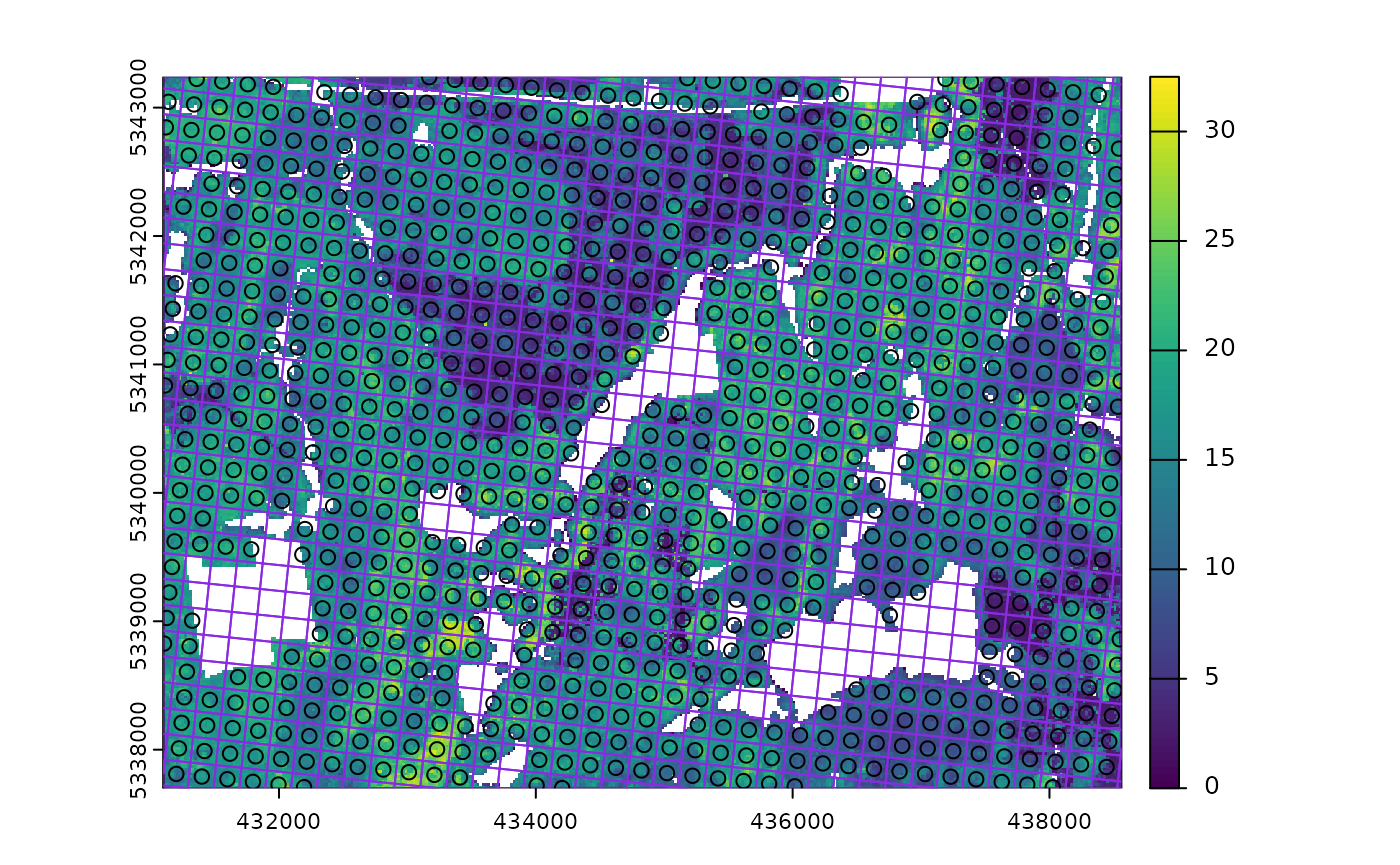Now lets sub-sample.

#--- sub sample using ---#
e <- existing %>%
extract_metrics(mraster = mraster, existing = .)

sample_existing(existing = e, nSamp = 300, plot = TRUE)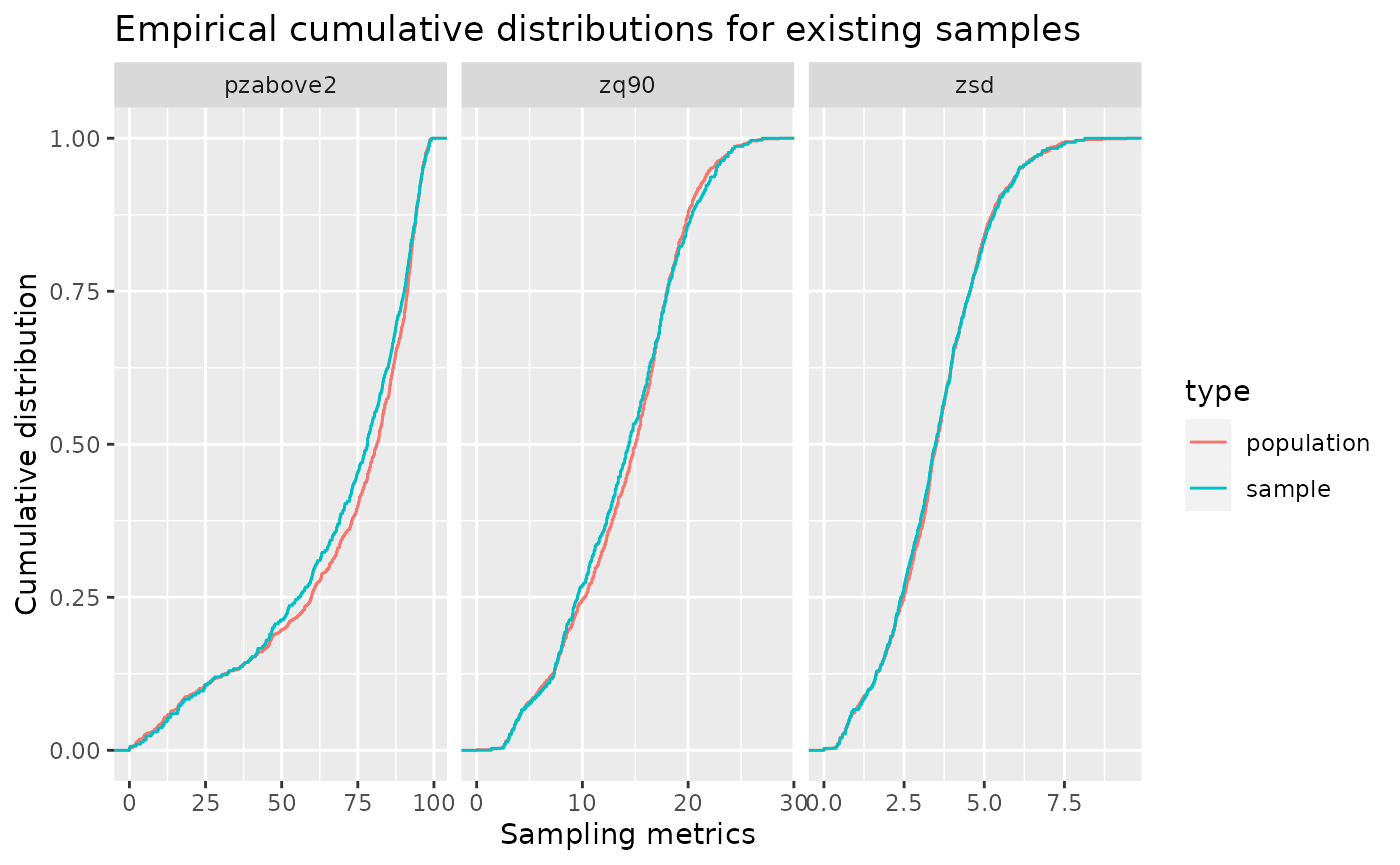#> Simple feature collection with 300 features and 3 fields
#> Geometry type: POINT
#> Dimension:     XY
#> Bounding box:  xmin: 431170 ymin: 5337710 xmax: 438490 ymax: 5343230
#> CRS:           +proj=utm +zone=17 +ellps=GRS80 +towgs84=0,0,0,0,0,0,0 +units=m +no_defs
#> First 10 features:
#>      zq90 pzabove2  zsd               geometry
#> 116 13.60     48.4 3.73 POINT (434090 5341670)
#> 737 14.60     83.4 3.98 POINT (437490 5341310)
#> 357 13.10     74.5 3.10 POINT (433790 5341670)
#> 260  5.25     83.2 0.98 POINT (434730 5342490)
#> 656 20.20     77.1 6.72 POINT (431450 5342890)
#> 536 15.10     15.9 4.17 POINT (435130 5340430)
#> 194 13.40     68.9 3.60 POINT (435150 5338730)
#> 861  8.06     49.7 2.11 POINT (435570 5338310)
#> 900 10.80     95.3 1.89 POINT (438010 5341210)
#> 818 16.00     87.7 4.18 POINT (437430 5341050)

TIP!

Notice that we used extract_metrics() after creating our existing. If the user provides a raster for the algorithm this isn’t neccesary (its done internally). If only sample units are given, attributes must be provided and sampling will be conducted on all included attributes.

We see from the output that we get 300 sample units that are a sub-sample of existing. The plotted output shows cumulative frequency distributions of the population (all existing samples) and the sub-sample (the 300 samples we requested).

### Sub-sampling using raster distributions

Our systematic sample of ~900 plots is fairly comprehensive, however we can generate a true population distribution through the inclusion of the ALS metrics in the sampling process. The metrics will be included in internal latin hypercube sampling to help guide sub-sampling of existing.

#--- sub sample using ---#
sample_existing(existing = existing, # our existing sample
nSamp = 300, # desired sample size
raster = mraster, # include mraster metrics to guide sampling of existing
plot = TRUE) # plot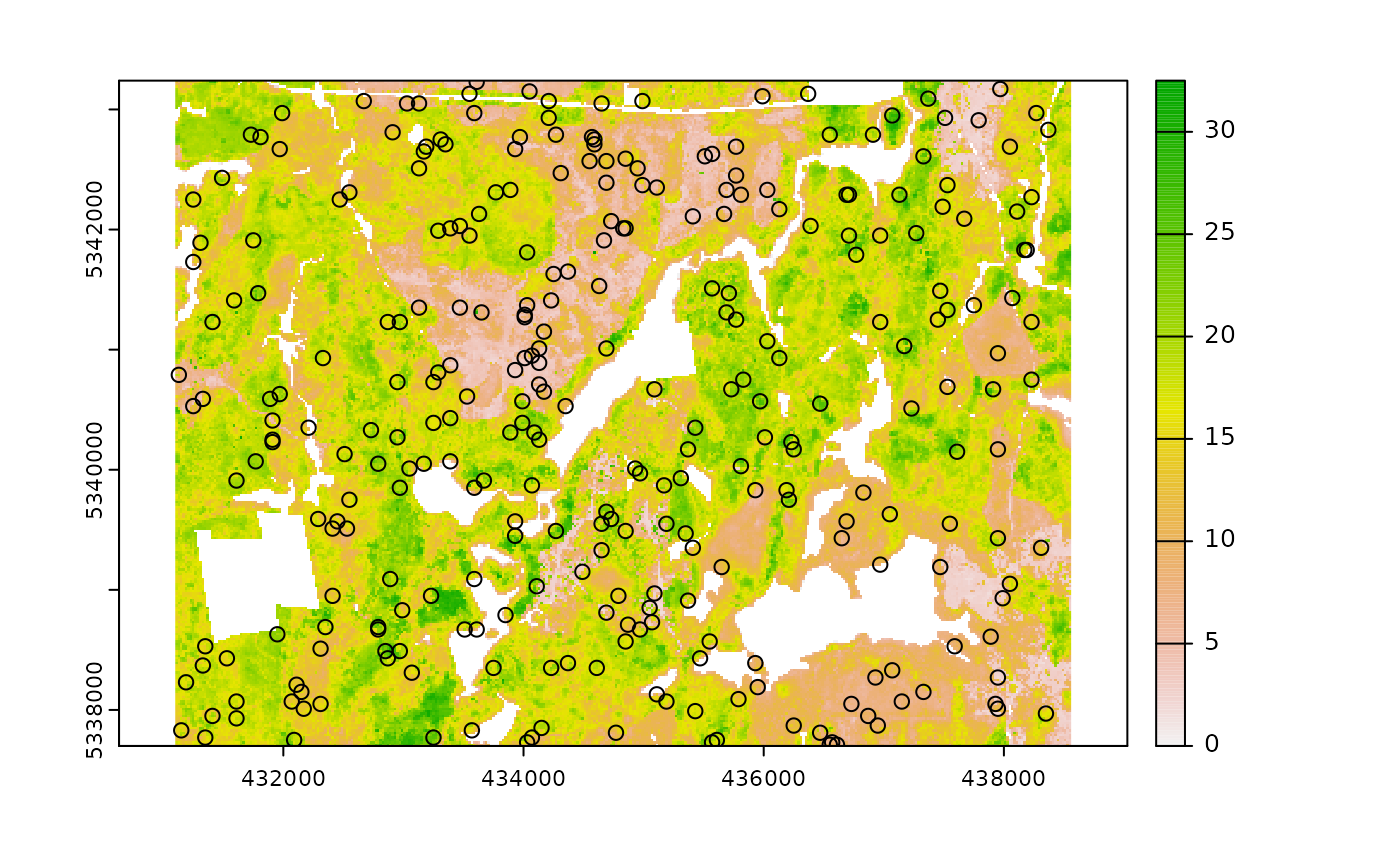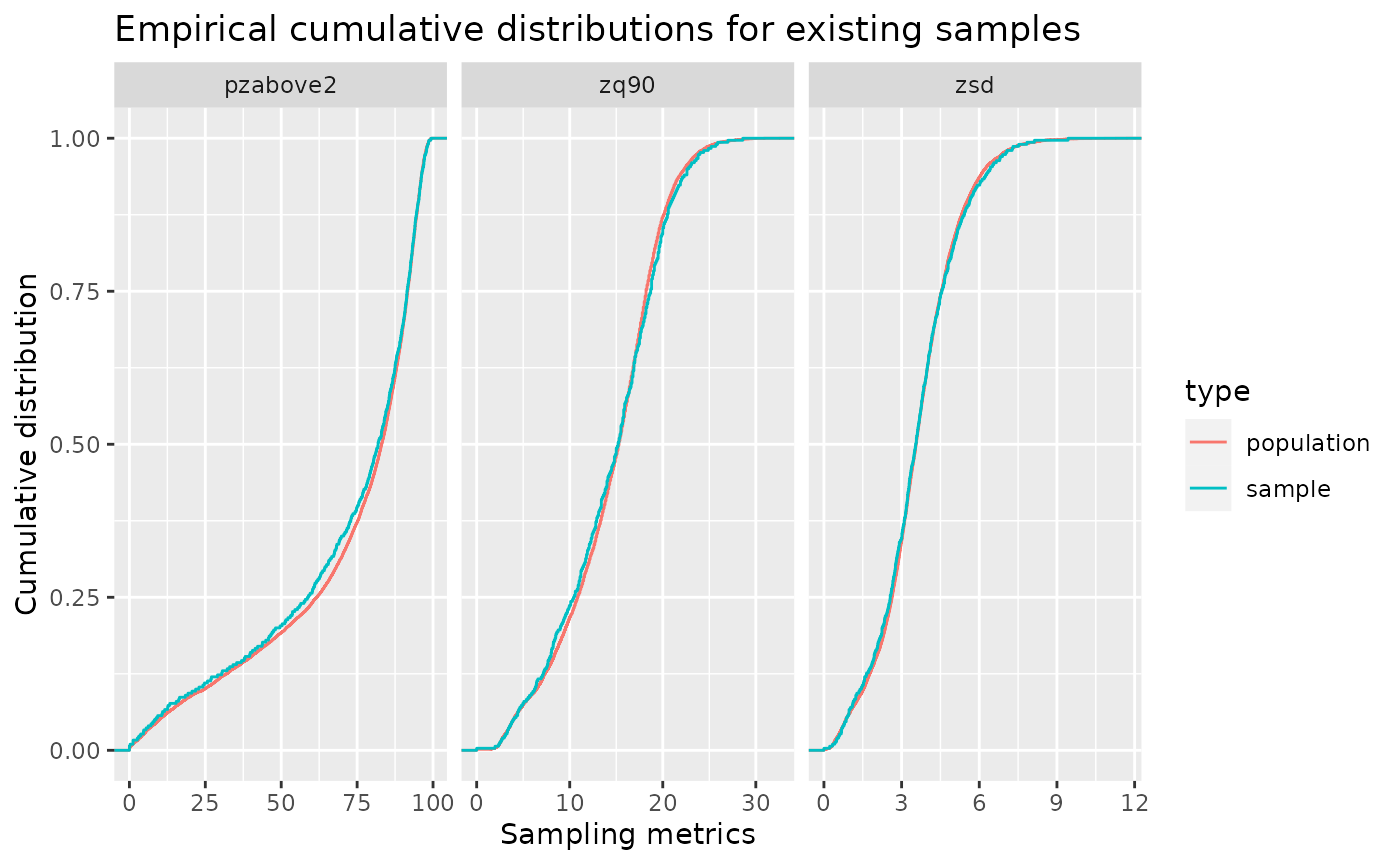#> Simple feature collection with 300 features and 3 fields
#> Geometry type: POINT
#> Dimension:     XY
#> Bounding box:  xmin: 431110 ymin: 5337710 xmax: 438490 ymax: 5343230
#> CRS:           +proj=utm +zone=17 +ellps=GRS80 +towgs84=0,0,0,0,0,0,0 +units=m +no_defs
#> First 10 features:
#>     zq90 pzabove2  zsd               geometry
#> 565 13.2     64.6 3.59 POINT (431370 5340650)
#> 130 11.3     40.8 3.16 POINT (435970 5342690)
#> 523 15.6     89.2 3.31 POINT (435410 5338130)
#> 431 12.2     59.7 3.50 POINT (435570 5338550)
#> 316 13.6     70.2 3.35 POINT (432630 5341390)
#> 358 14.6     63.2 3.92 POINT (437850 5341330)
#> 78  13.1     69.7 3.42 POINT (437490 5340550)
#> 731 19.1     96.9 4.18 POINT (434770 5338550)
#> 188 16.0     55.0 4.91 POINT (435790 5339690)
#> 820 10.3     39.6 2.83 POINT (435030 5341650)

The sample distribution again mimics the population distribution quite well! Now lets try using a cost variable to constrain the sub-sample.

#--- create distance from roads metric ---#
dist <- calculate_distance(raster = mraster, access = access)
#>
|---------|---------|---------|---------|
=========================================

#--- sub sample using ---#
sample_existing(existing = existing, # our existing sample
nSamp = 300, # desired sample size
raster = dist, # include mraster metrics to guide sampling of existing
cost = 4, # either provide the index (band number) or the name of the cost layer
plot = TRUE) # plot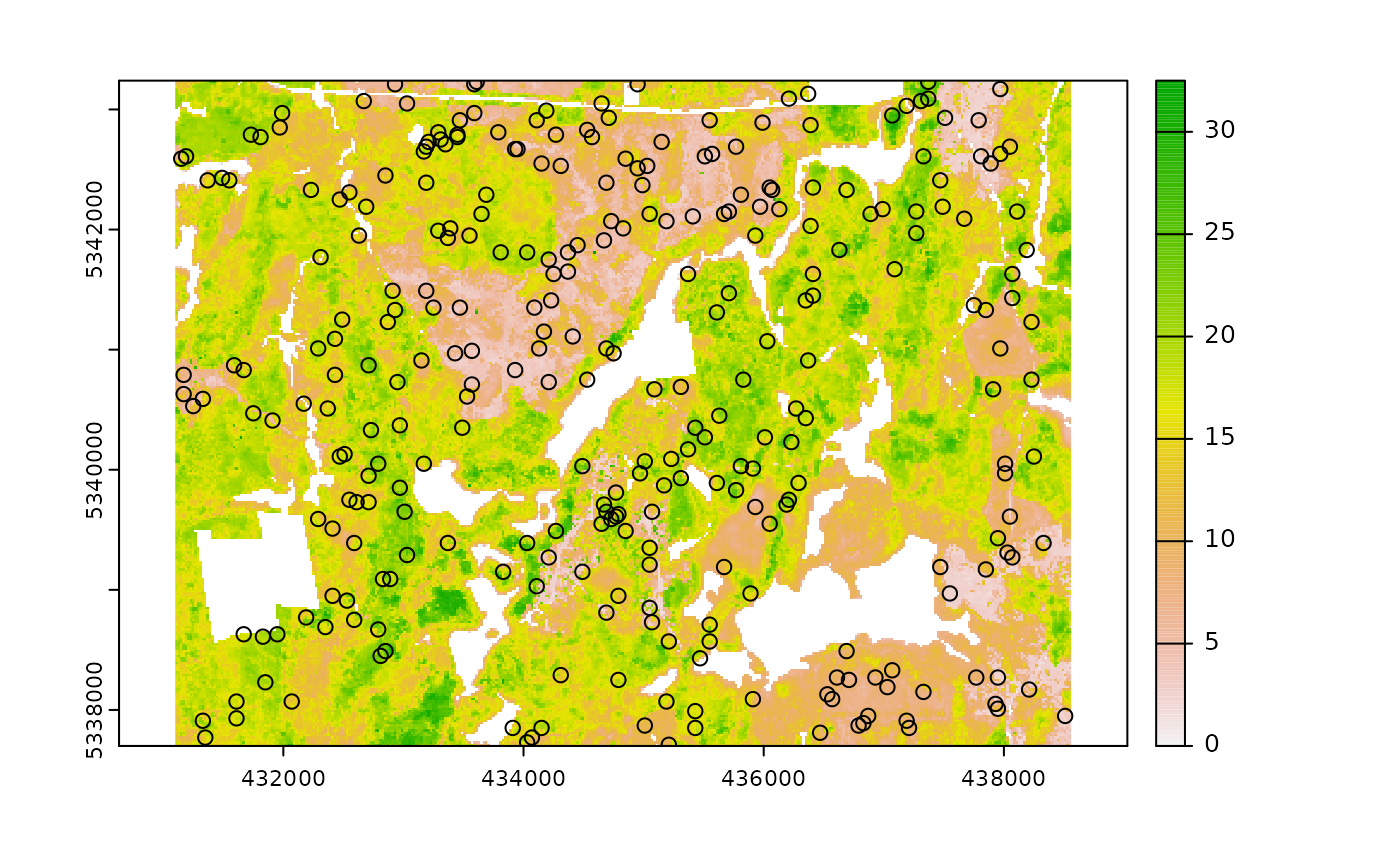#> Simple feature collection with 300 features and 4 fields
#> Geometry type: POINT
#> Dimension:     XY
#> Bounding box:  xmin: 431110 ymin: 5337710 xmax: 438490 ymax: 5343230
#> CRS:           +proj=utm +zone=17 +ellps=GRS80 +towgs84=0,0,0,0,0,0,0 +units=m +no_defs
#> First 10 features:
#>      zq90 pzabove2  zsd dist2access               geometry
#> 238 17.60     85.9 4.82   310.32707 POINT (436590 5340910)
#> 717  4.65     48.8 1.01   164.75811 POINT (435210 5342810)
#> 402 20.30     88.2 6.06    66.28027 POINT (437250 5341850)
#> 56  13.40     88.8 3.04   342.15879 POINT (436490 5341910)
#> 438 23.40     95.3 5.28   264.63964 POINT (435650 5337870)
#> 726 21.00     85.3 4.57   435.86532 POINT (436730 5340810)
#> 699  9.59     42.9 2.18     3.01315 POINT (436810 5341550)
#> 823 18.30     89.0 5.45    20.68297 POINT (433390 5342730)
#> 61  13.00     82.7 3.58   136.72996 POINT (432650 5338630)
#> 883 12.00     94.7 2.57   395.59118 POINT (438390 5341790)

Finally, should the user wish to further constrain the sample based on access like other sampling approaches in sgsR that is also possible.

#--- ensure access and existing are in the same CRS ---#

sf::st_crs(existing) <- sf::st_crs(access)

#--- sub sample using ---#
sample_existing(existing = existing, # our existing sample
nSamp = 300, # desired sample size
raster = dist, # include mraster metrics to guide sampling of existing
cost = 4, # either provide the index (band number) or the name of the cost layer
access = access, # roads layer
buff_inner = 50, # inner buffer - no sample units within this distance from road
buff_outer = 300, # outer buffer - no sample units further than this distance from road
plot = TRUE) # plot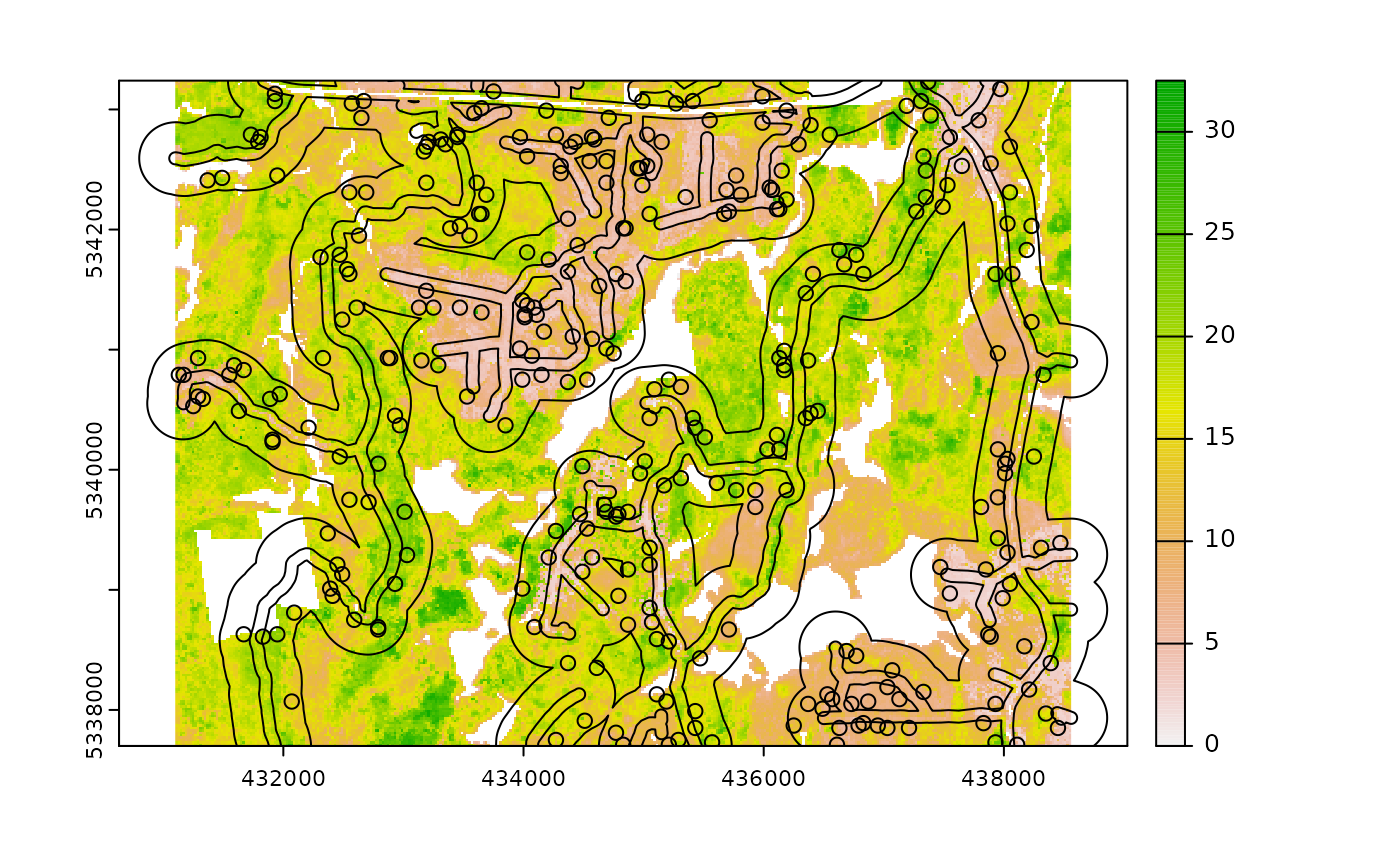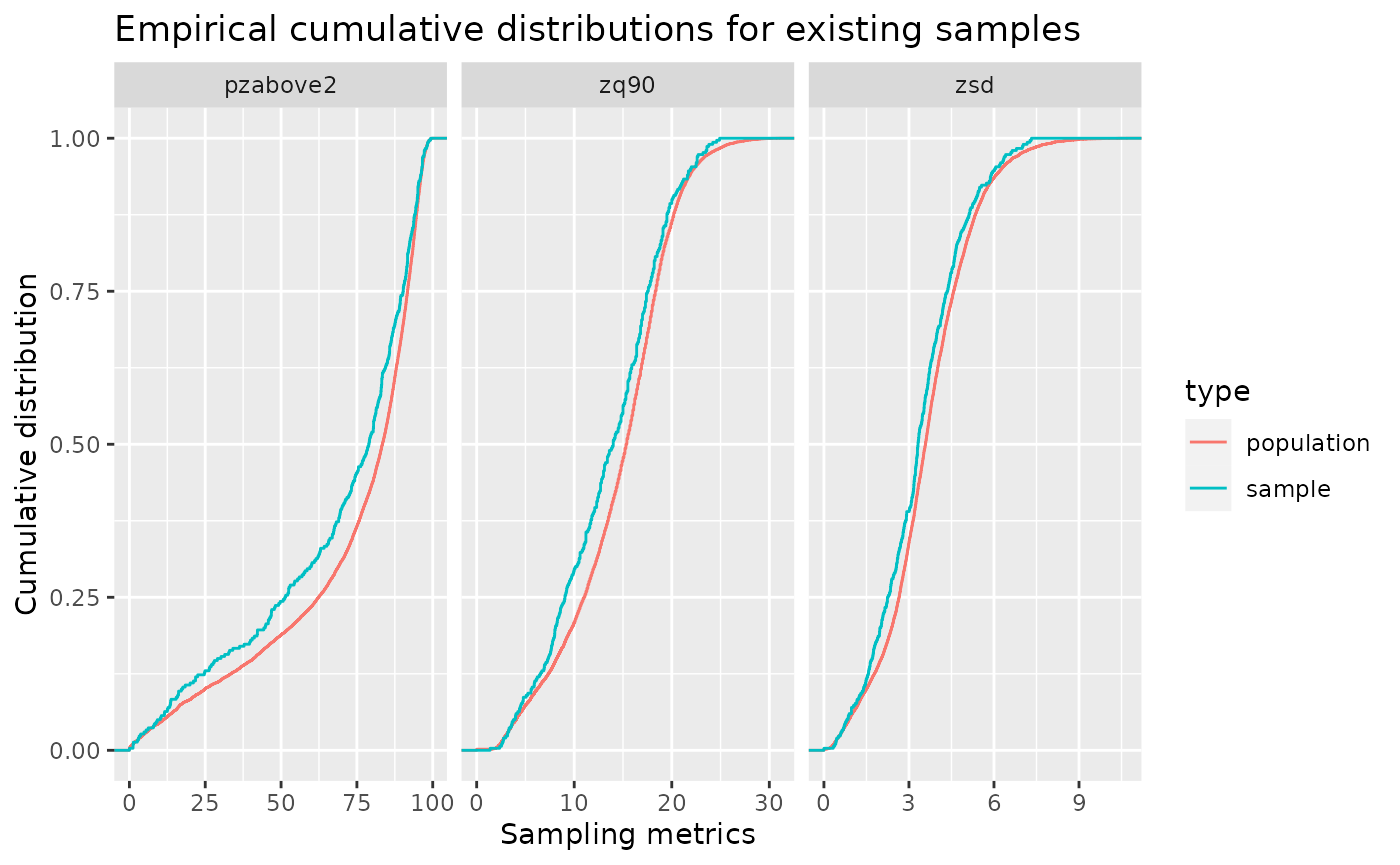#> Simple feature collection with 300 features and 4 fields
#> Geometry type: POINT
#> Dimension:     XY
#> Bounding box:  xmin: 431110 ymin: 5337710 xmax: 438490 ymax: 5343230
#> Projected CRS: UTM_Zone_17_Northern_Hemisphere
#> First 10 features:
#>      zq90 pzabove2  zsd dist2access               geometry
#> 134 21.10     93.3 4.20   140.83325 POINT (431830 5340610)
#> 379 11.30     23.2 3.37   199.59164 POINT (438030 5342510)
#> 45  12.80     69.0 3.28   131.83490 POINT (435890 5339170)
#> 139  6.10     34.6 1.55    82.78292 POINT (434550 5341630)
#> 326 16.50     53.6 5.00   168.30334 POINT (432710 5341550)
#> 65  15.80     94.8 3.75   104.28371 POINT (434950 5342590)
#> 28   3.99     67.2 0.78    56.25403 POINT (437550 5343050)
#> 376 14.70     92.0 3.13    87.64066 POINT (432370 5340290)
#> 404 12.70     95.1 2.71    58.71828 POINT (435850 5342150)
#> 420 12.00     88.6 2.43    77.97024 POINT (438070 5340230)`

TIP!

The greater constraints we add to sampling, the less likely we will have strong correlations between the population and sample, so its always important to understand these limitations and plan accordingly.